X
Typical Bar Bends
Shape Code 00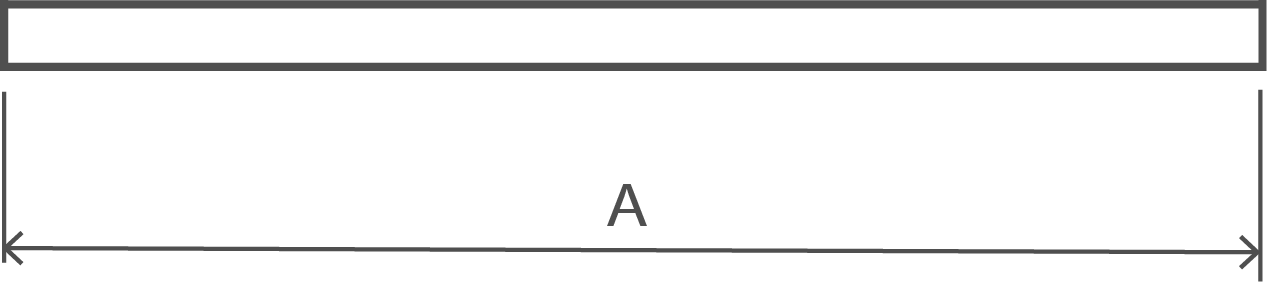A

Shape Code 01A - Stock Lengths. See Note 4.

Shape Code 11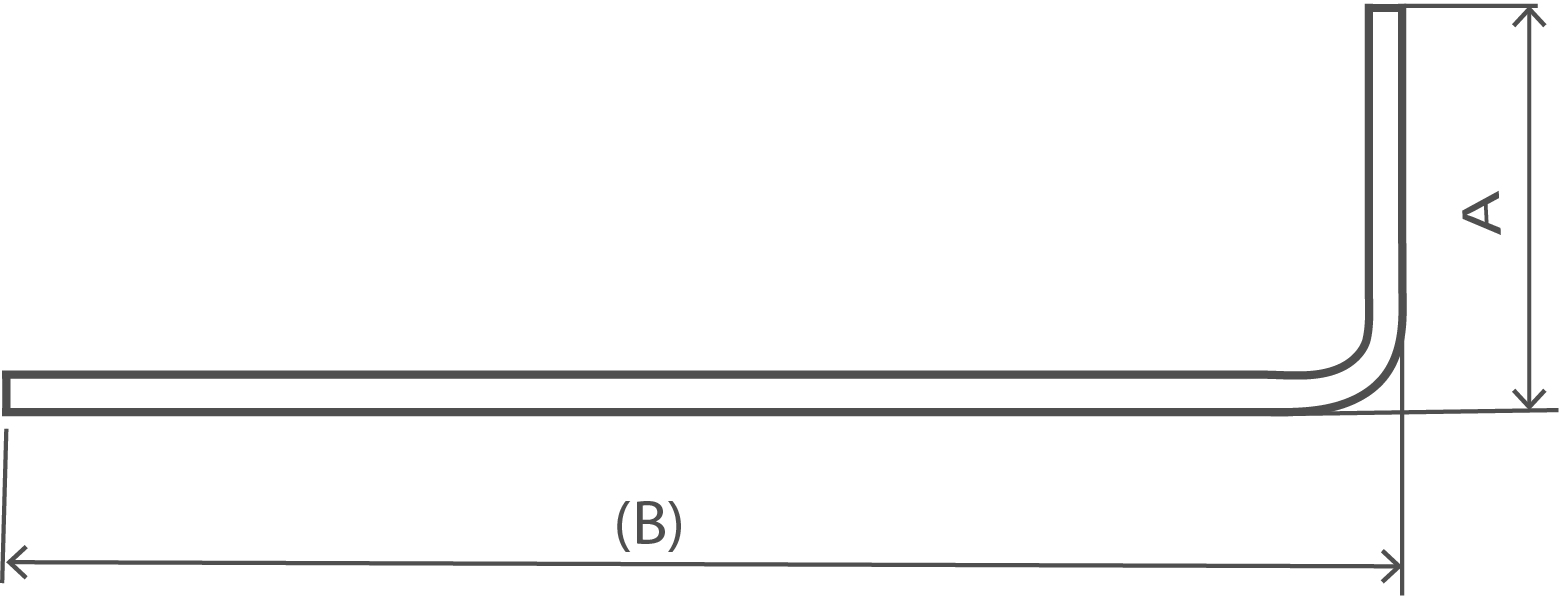A + (B) - 0.5r - d

Shape Code 12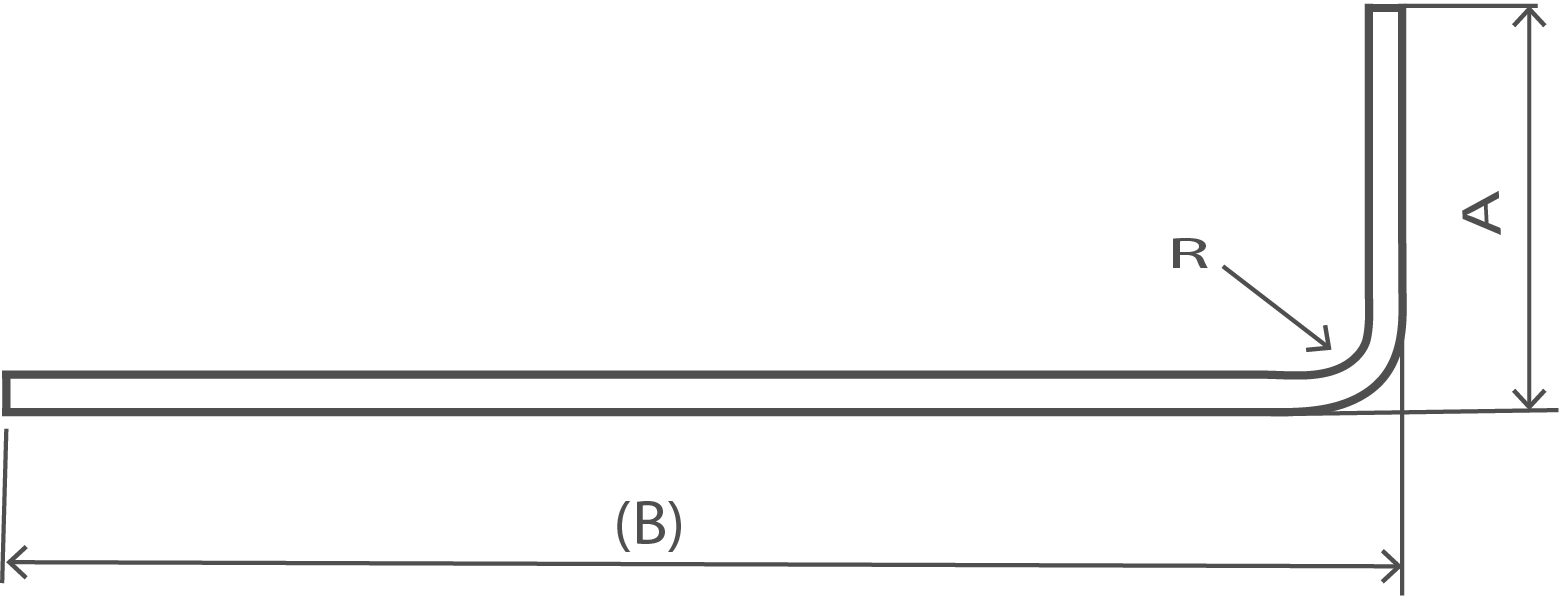A + (B) - 0.43R - 1.2d Neither A nor B shall be less than (R+6d)

Shape Code 13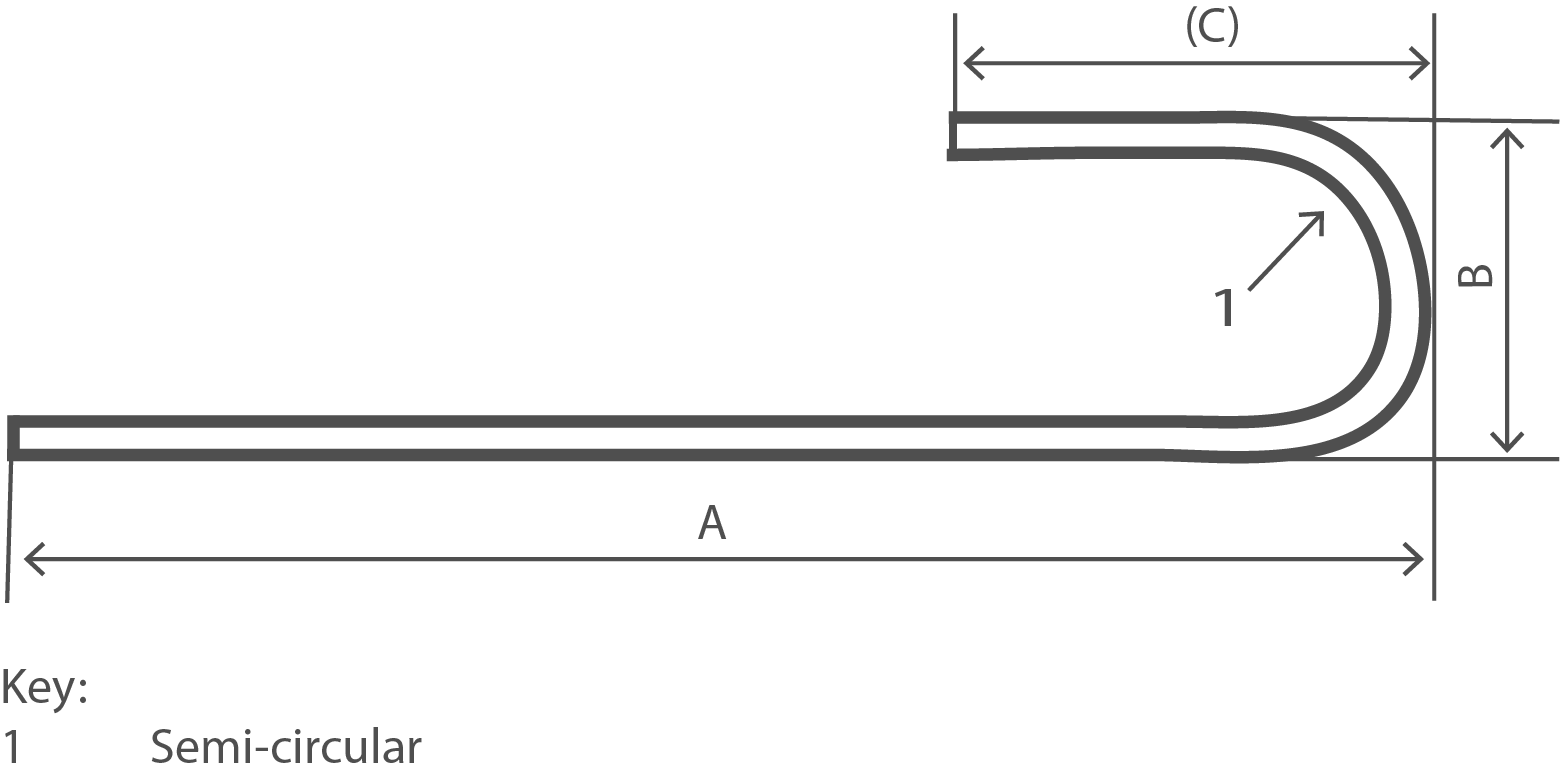A + 0.57B + (C) - 1.6d B shall not be less than 2(r+d)

Shape Code 14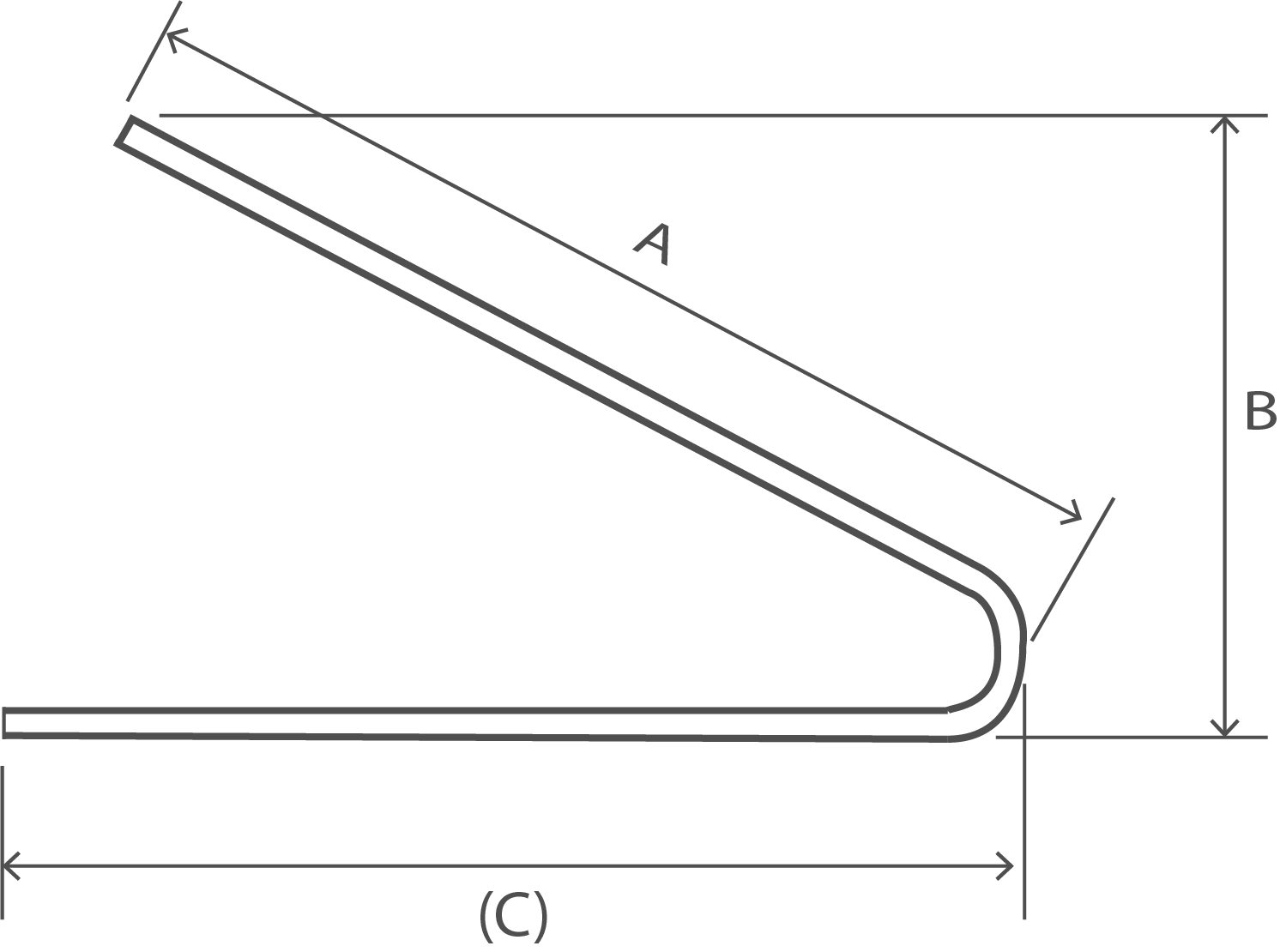A + (C) - 4d

Shape Code 15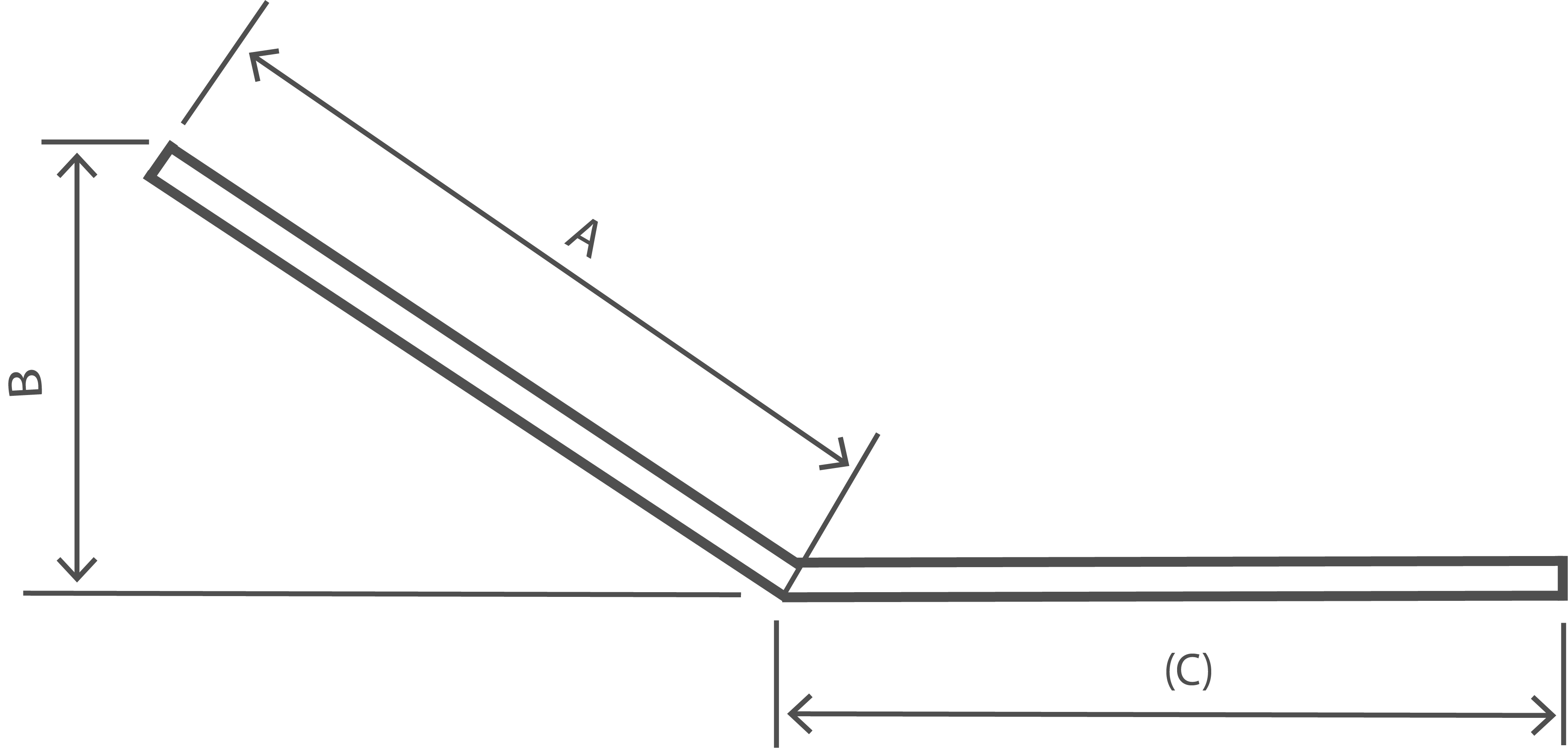A + (C)

Shape Code 21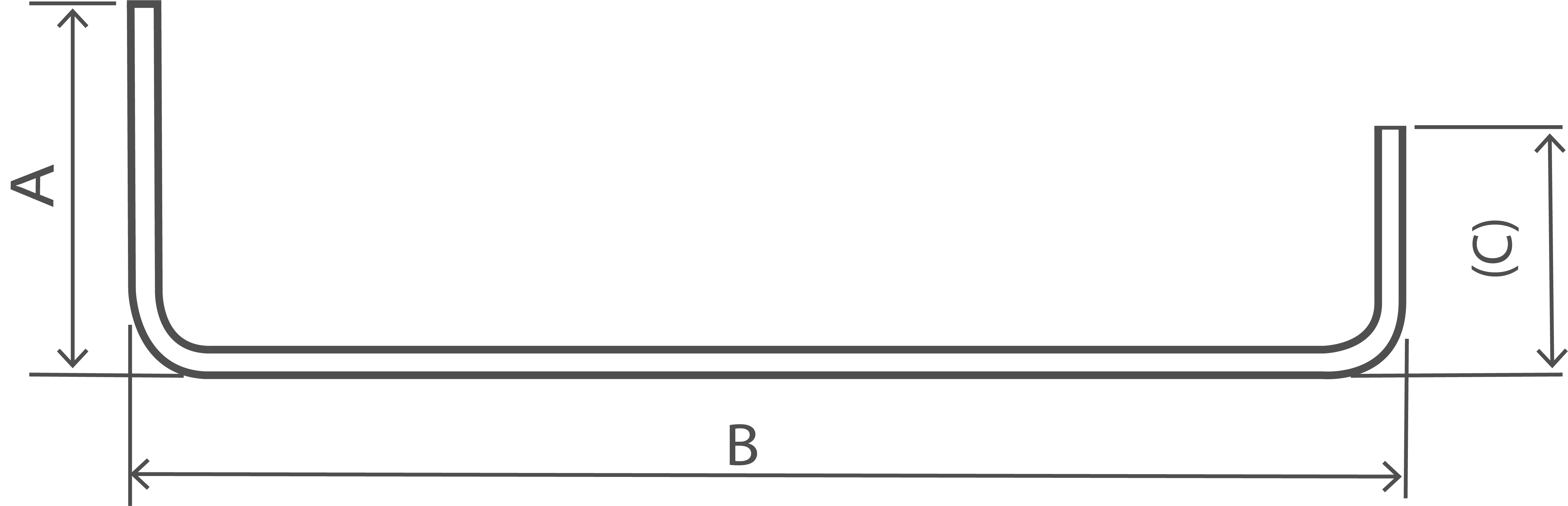A + B + (C) - r - 2d

Shape Code 22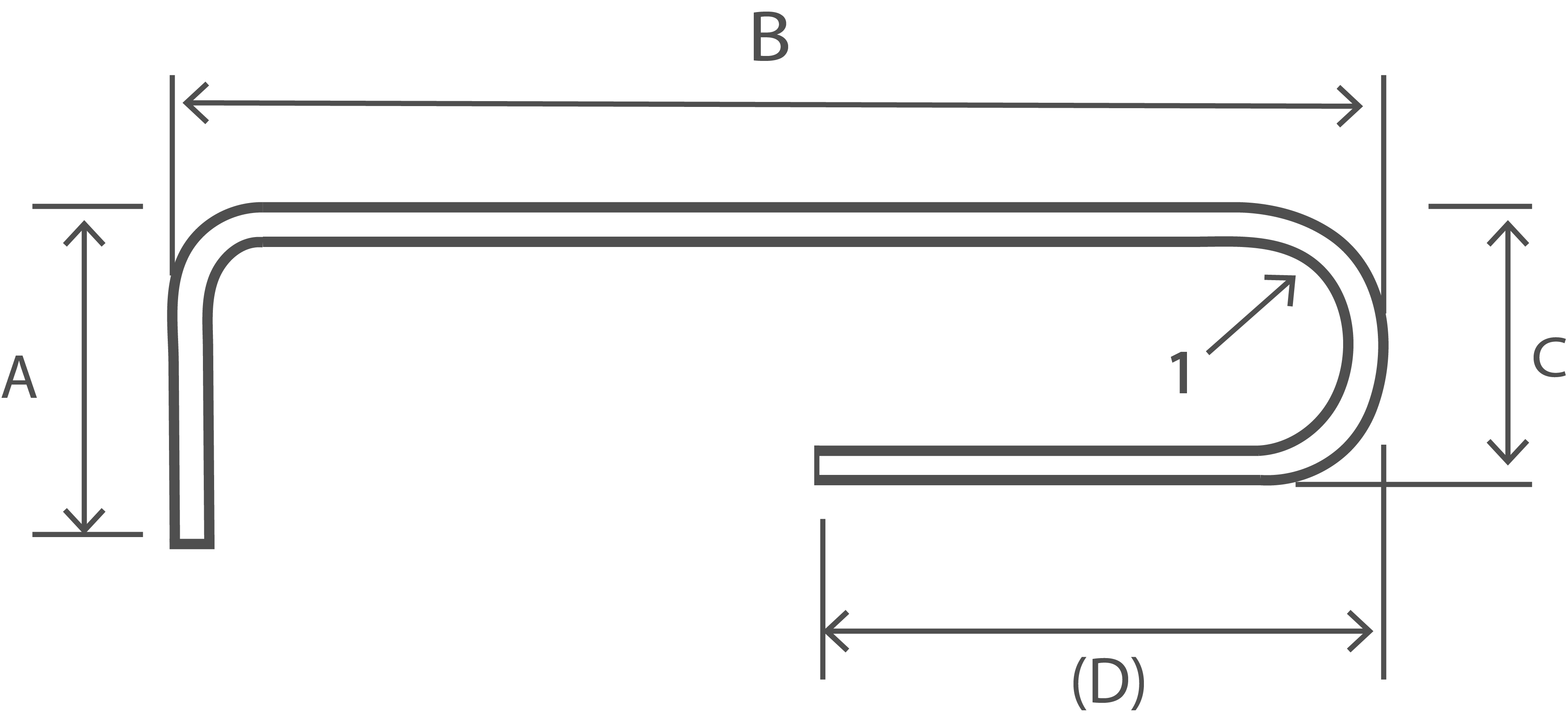A + B + C + (D) - 1.5r - 3d C shall not be less than 2(r+d). (D) shall not be less than C/2 +5d

Shape Code 23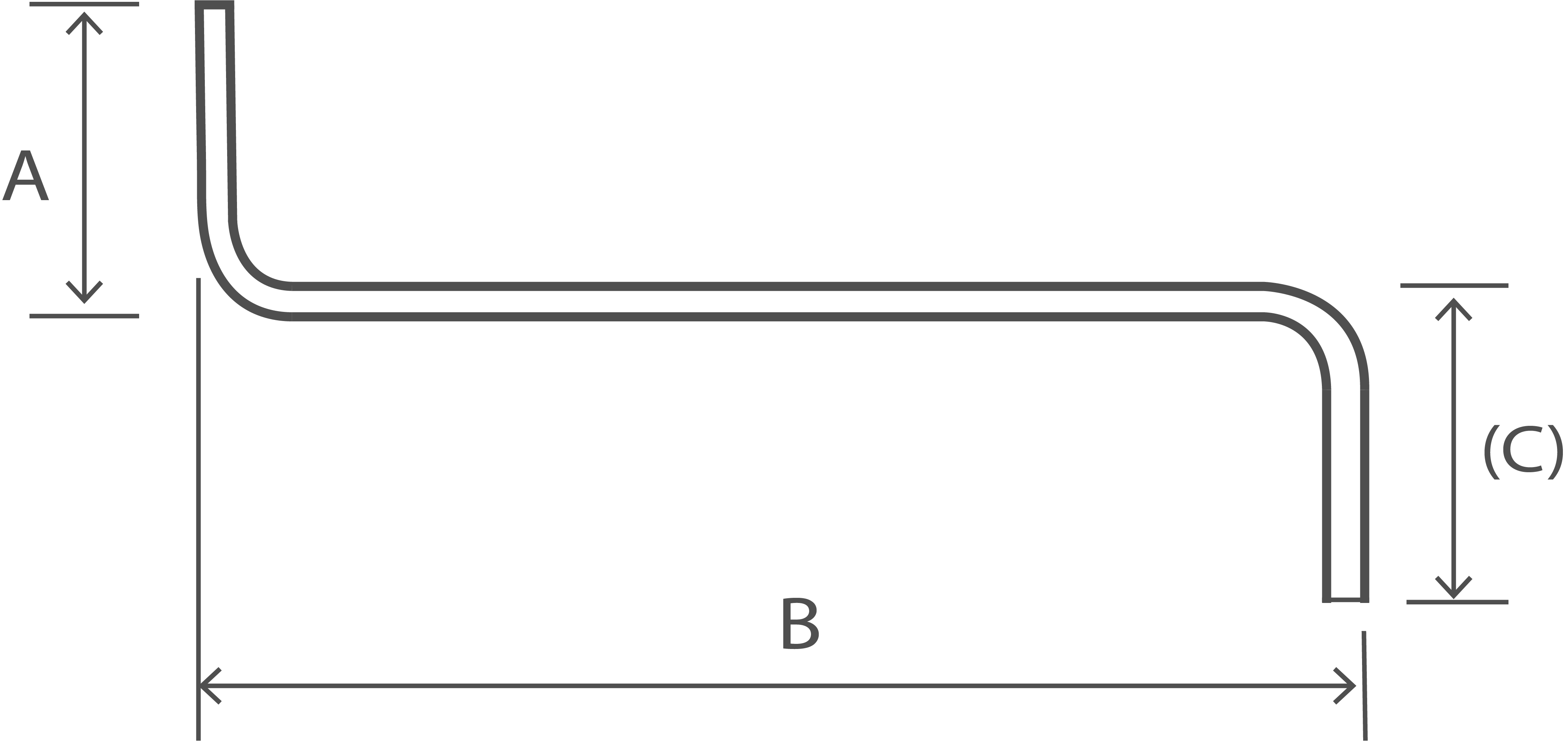A + B + (C) - r - 2d

Shape Code 24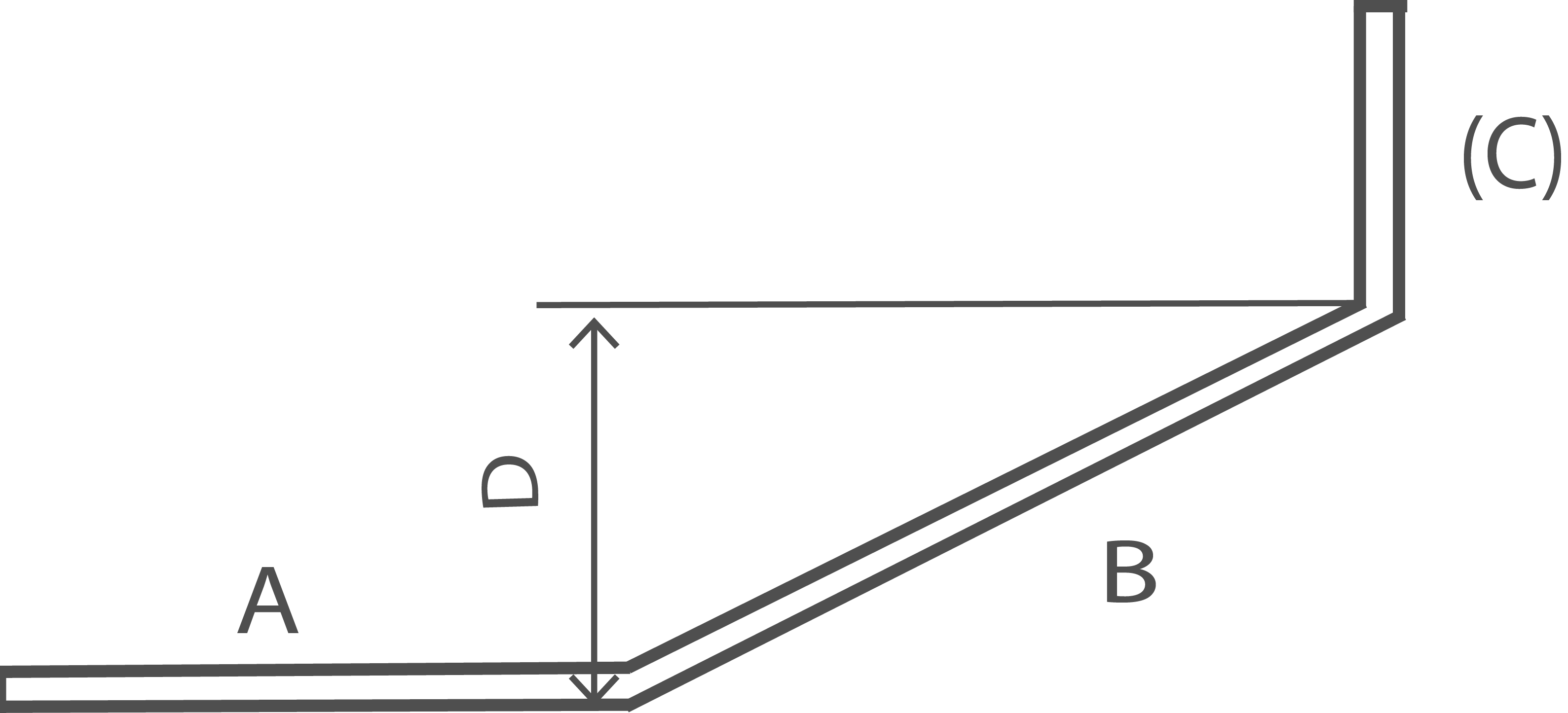A + B + (C) A and (C) are at 90° to one another

Shape Code 25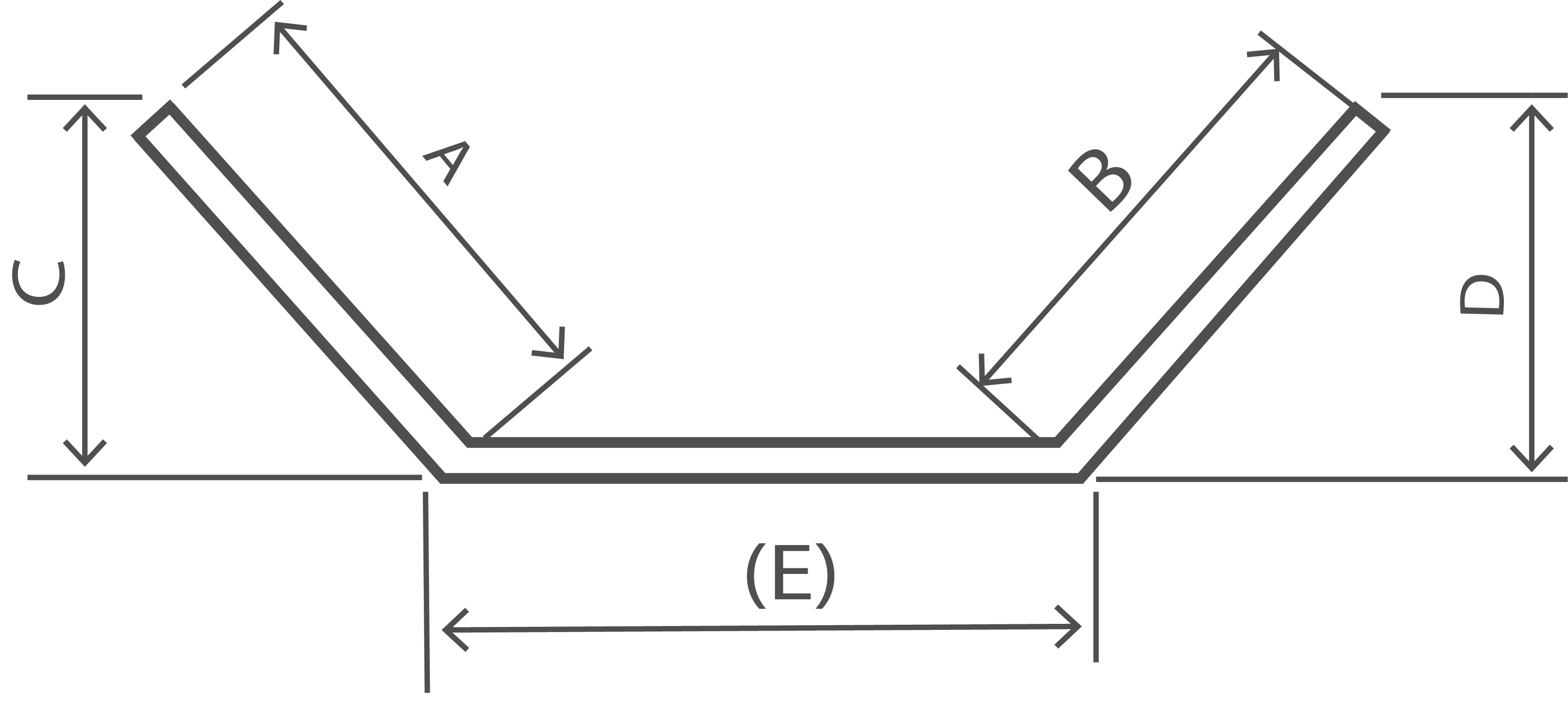A + B + (E) If E is the critical dimension, schedule a 99 and specify A or B as the free dimension. See Note 1.

Shape Code 26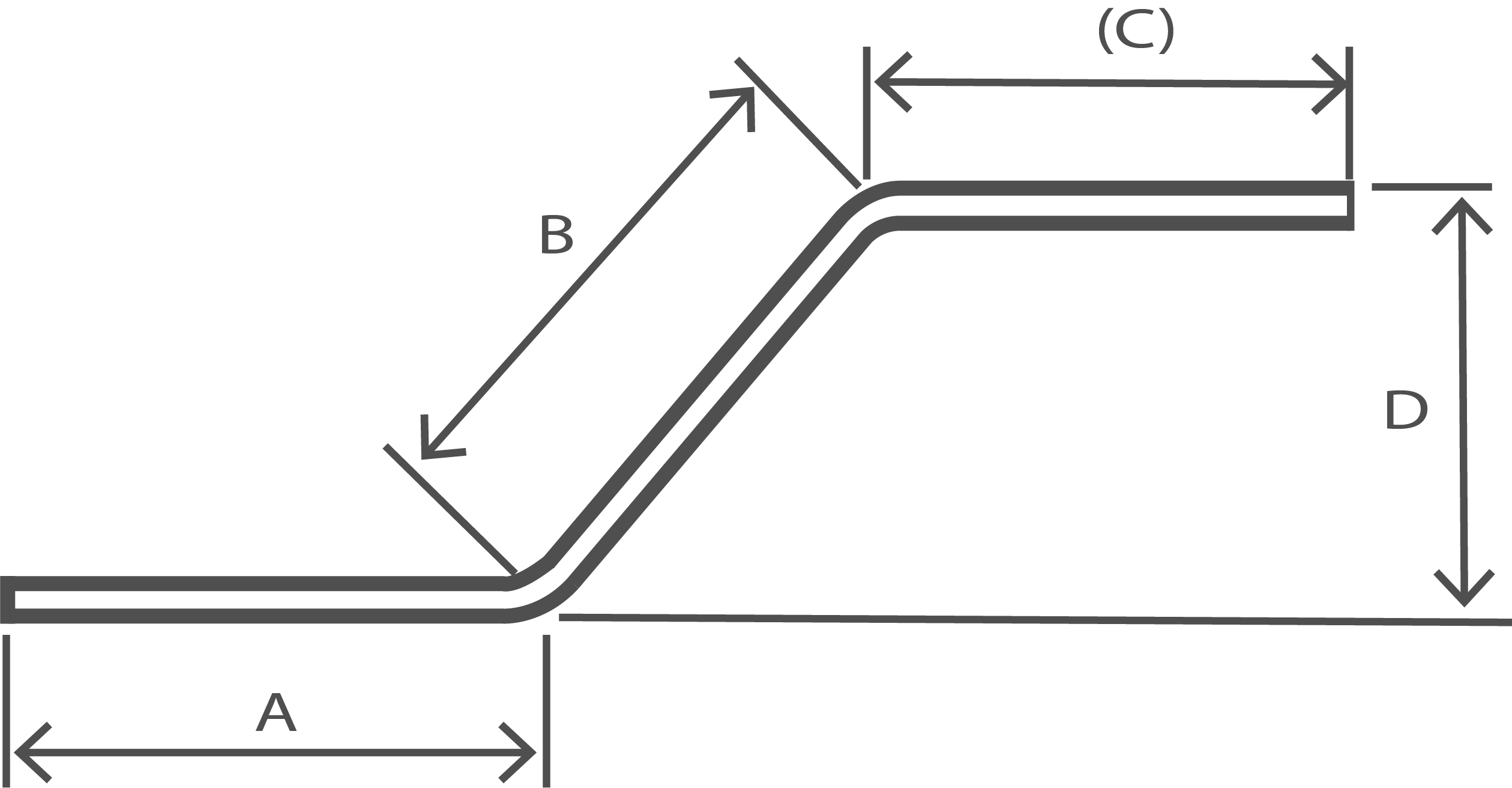A + B + (C)

Shape Code 27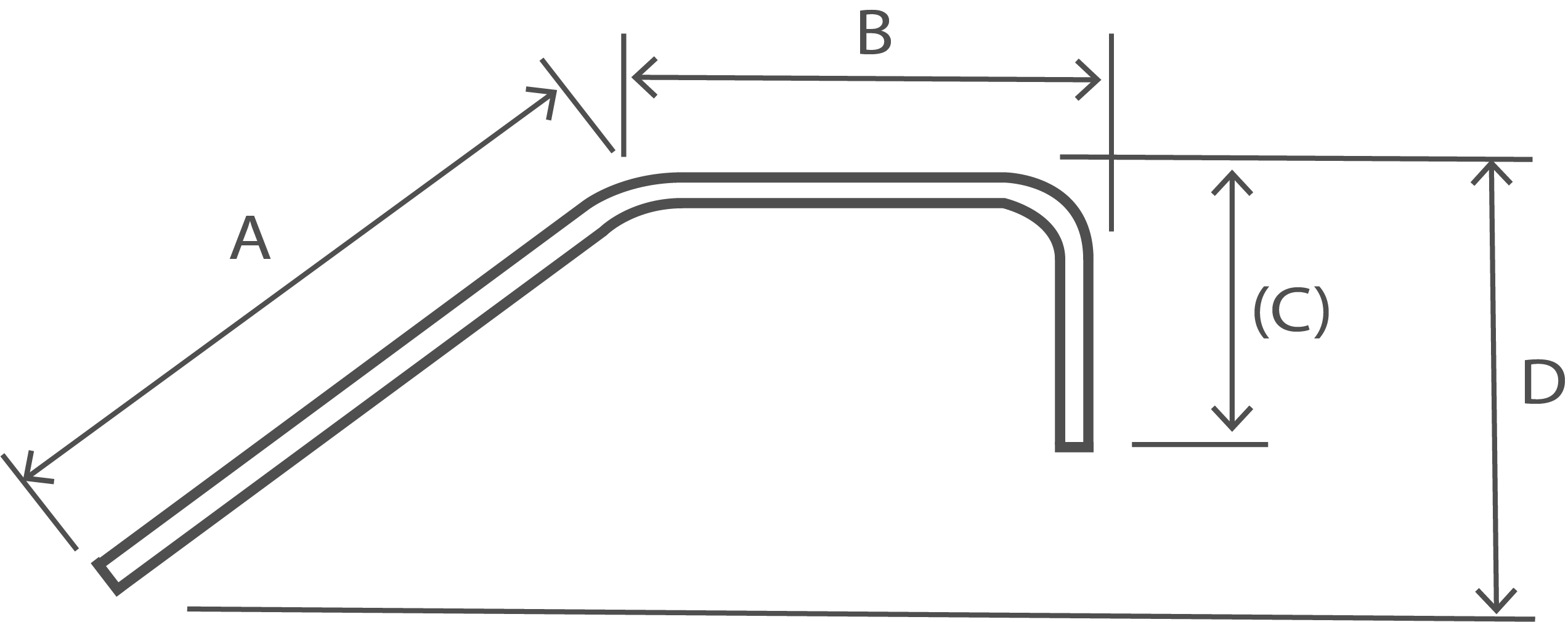A + B + (C) - 0.5r - d

Shape Code 28A + B + (C) - 0.5r - d If E is the critical dimension, schedule a 99 and specify A or B as the free dimension. See Note 1.

Shape Code 29A + B + C + (D) - r - 2d

Shape Code 31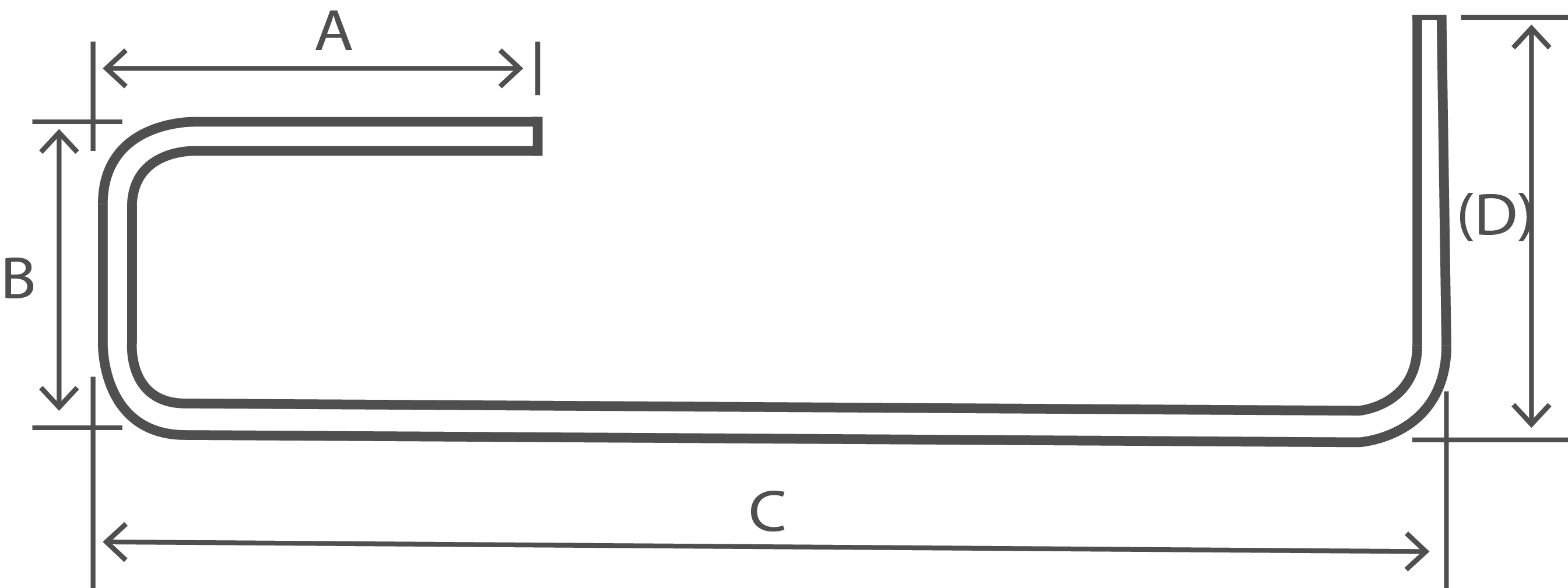A + B + C + (D) - 1.5r - 3d

Shape Code 32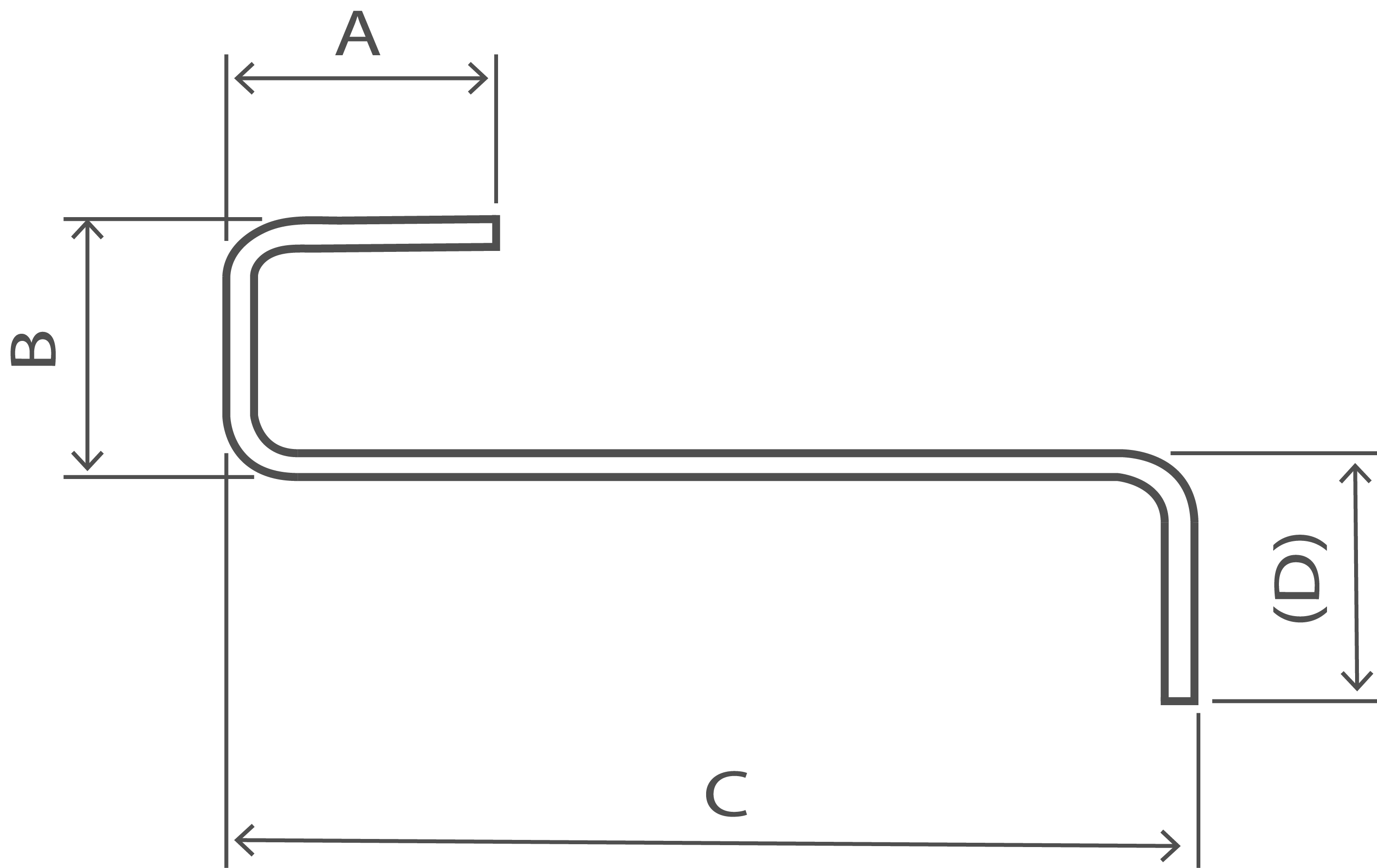A + B + C + (D) - 1.5r - 3d If E is the critical dimension, schedule a 99 and specify A or B as the free dimension. See Note 1.

Shape Code 33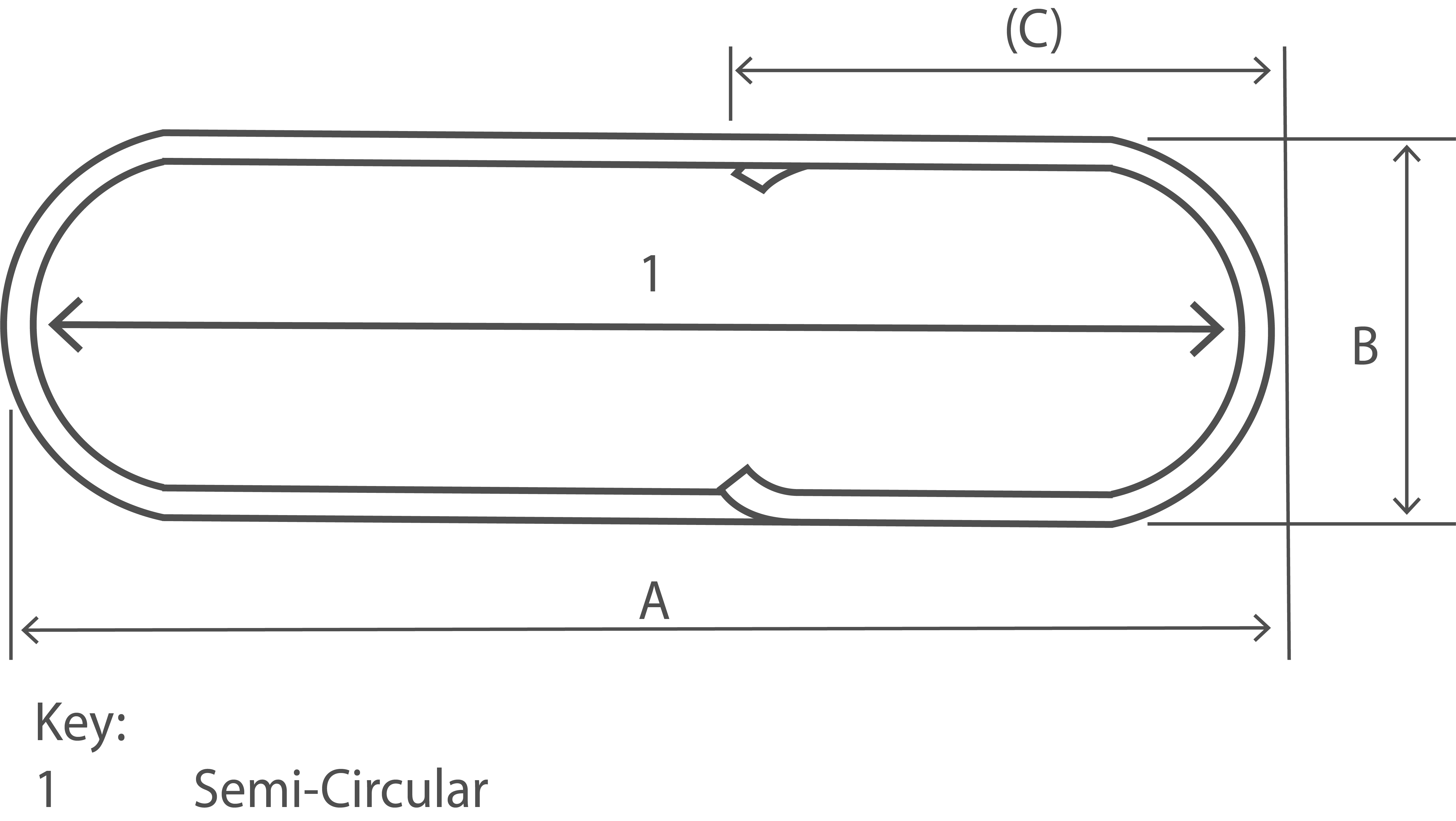2A + 1.7B + 2(C) - 4d A shall not be less than 12d + 30 B shall not be less than 2(r + d)/p> (C) shall not be less than B/2 + 5d See Note 3

Shape Code 34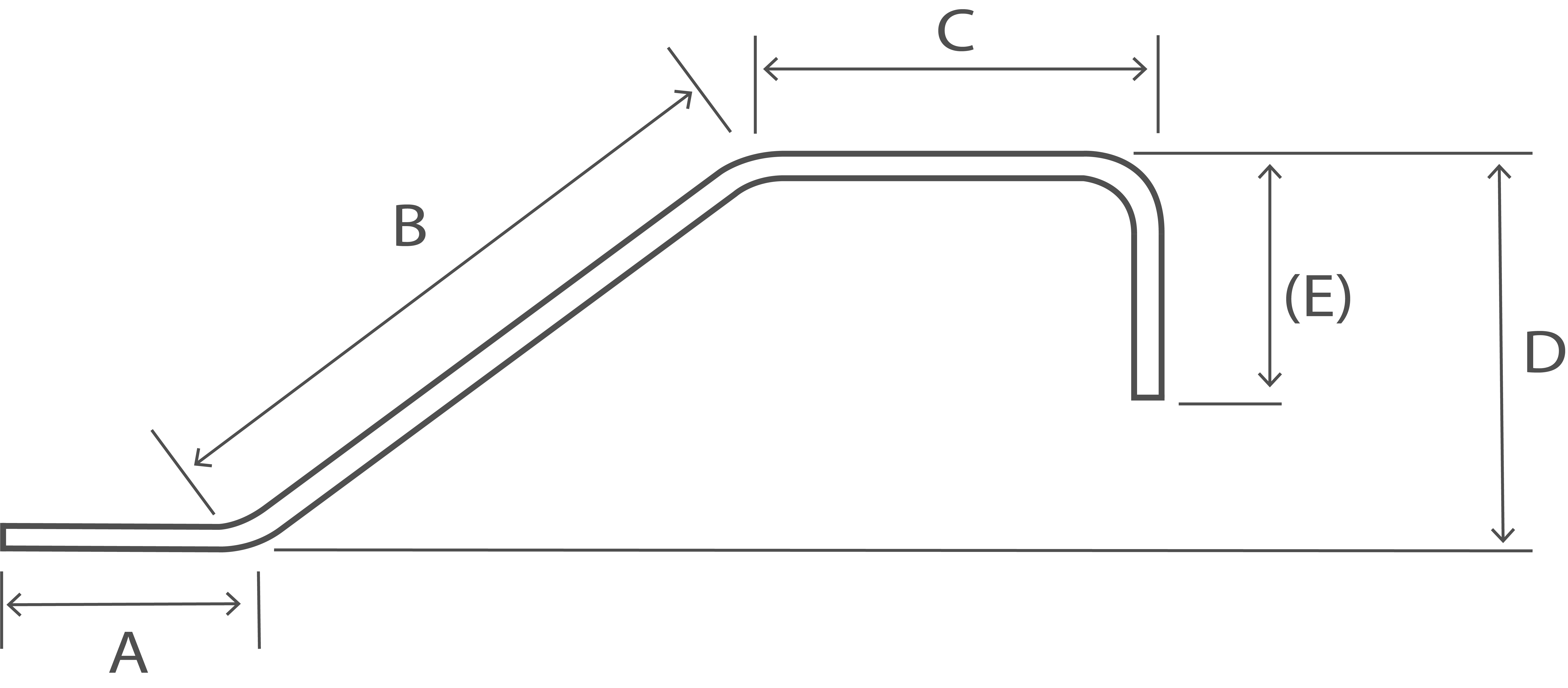A + B + C + (E) - 0.5r - d See Note 1

Shape Code 35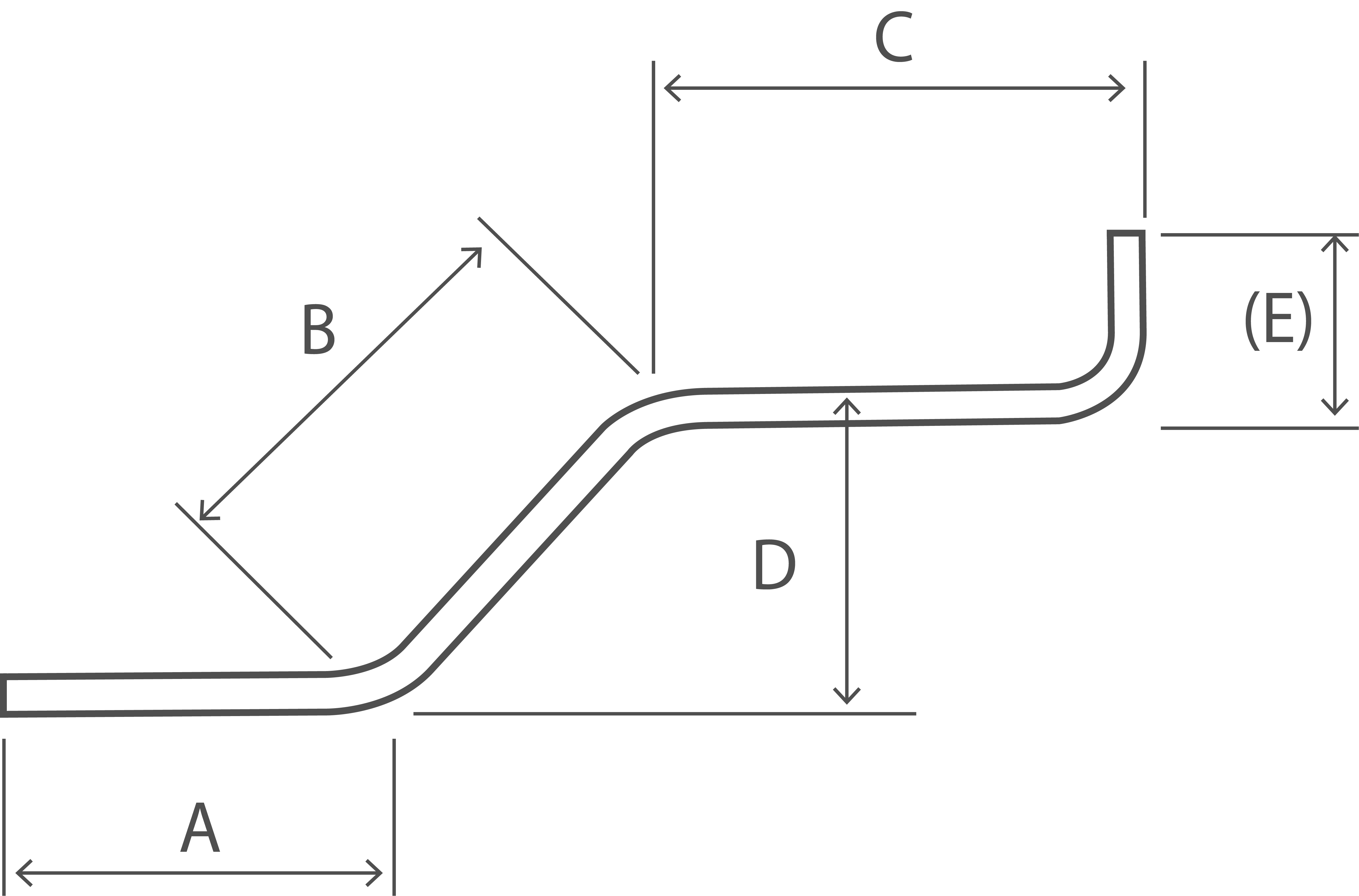A + B + C + (E) - 0.5r - d See Note 1

Shape Code 36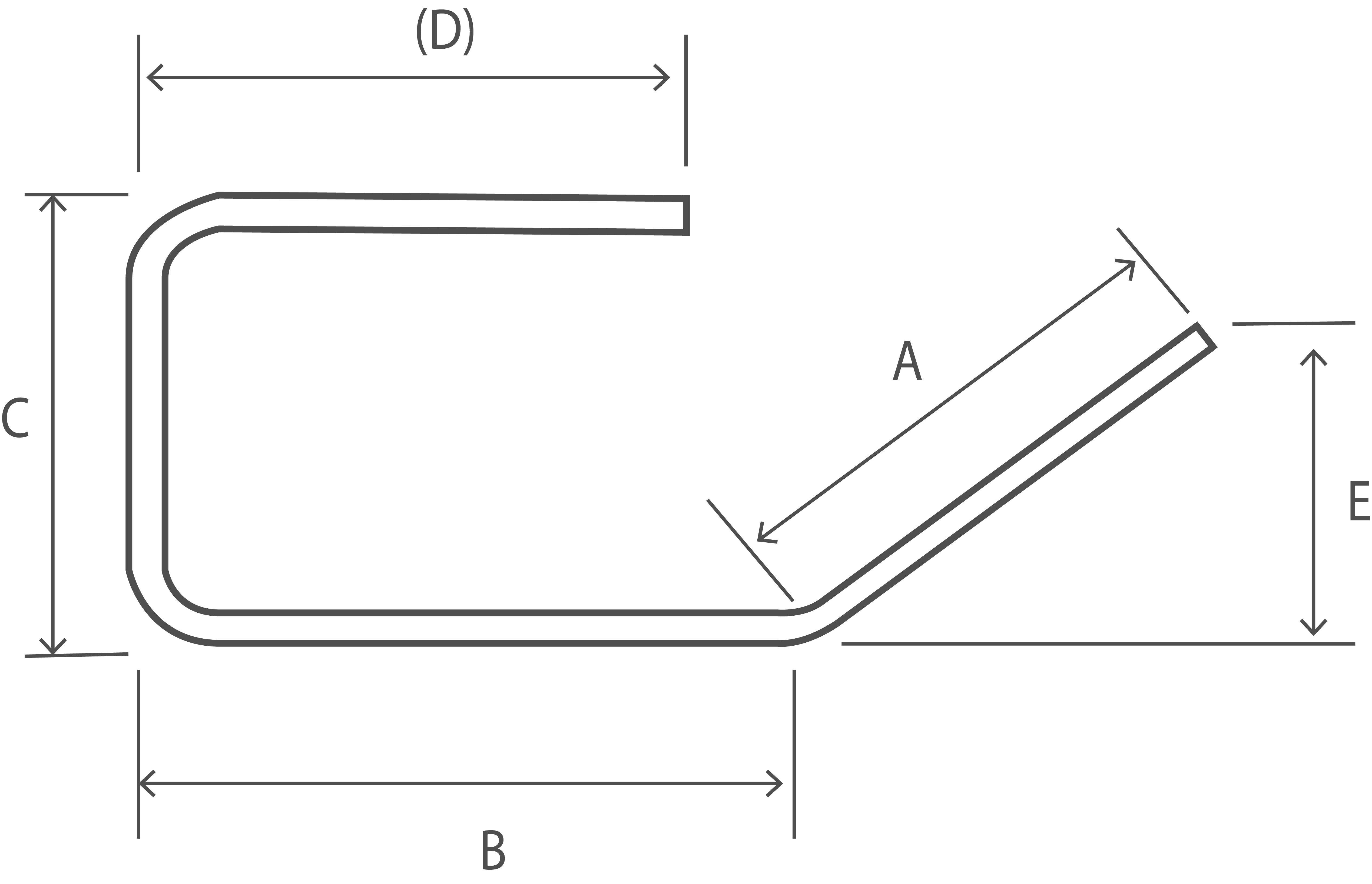A + B + C + (D) - r - 2d See Note 1

Shape Code 41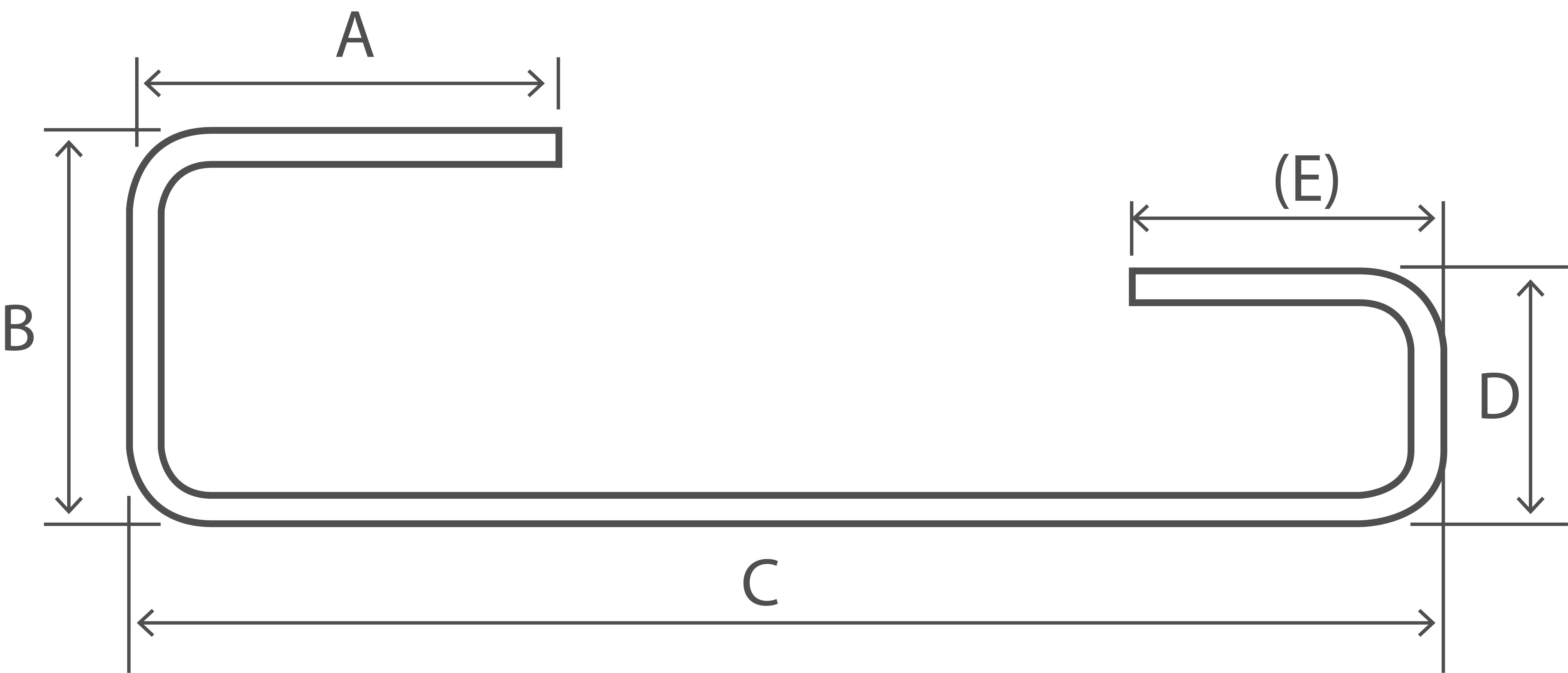A + B + C + D + (E) - 2r - 4d May also be used for flag link viz: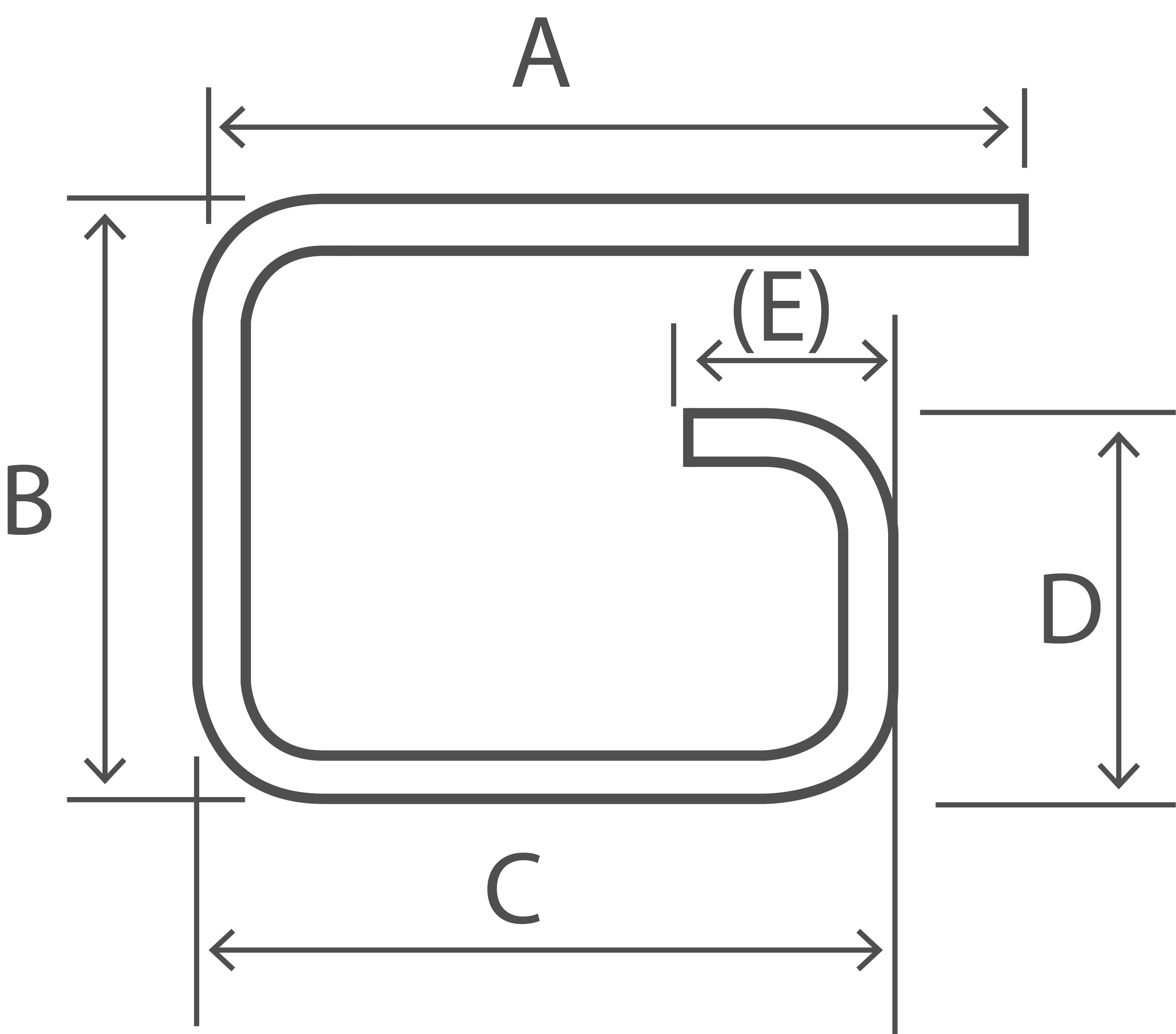Shape Code 44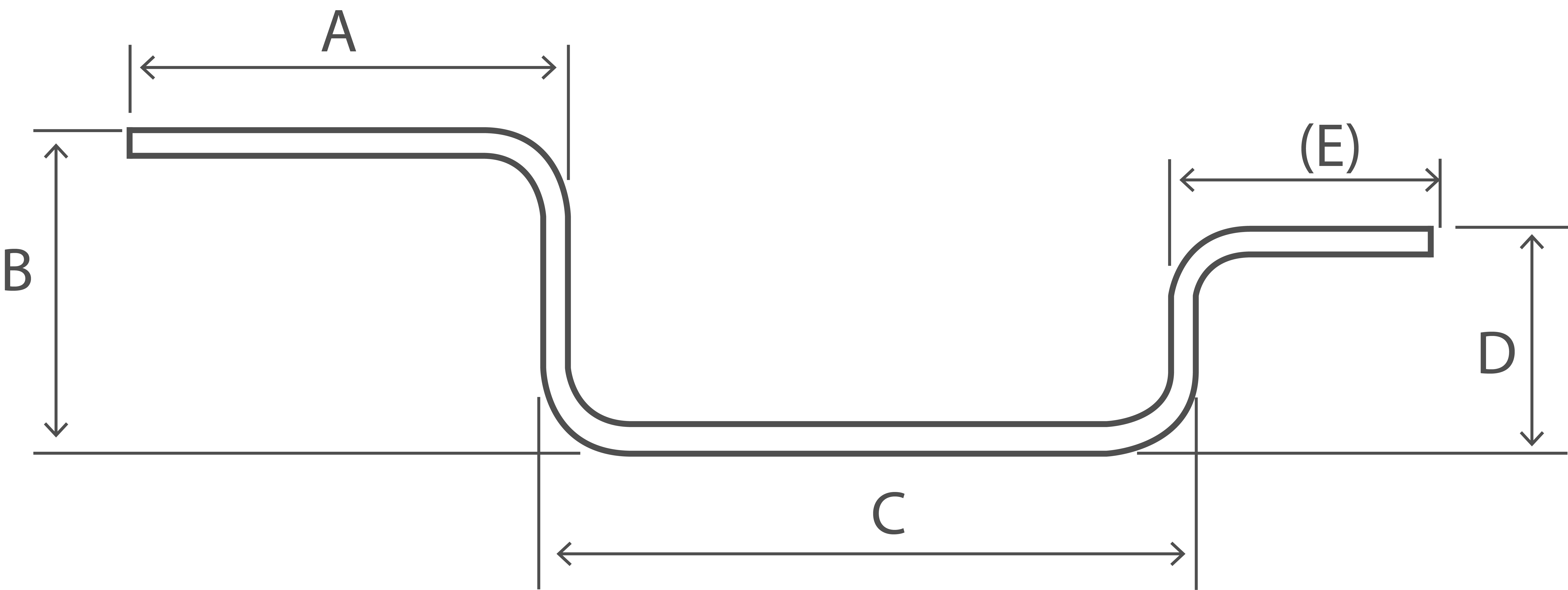A + B + C + D + (E) - 2r - 4d

Shape Code 46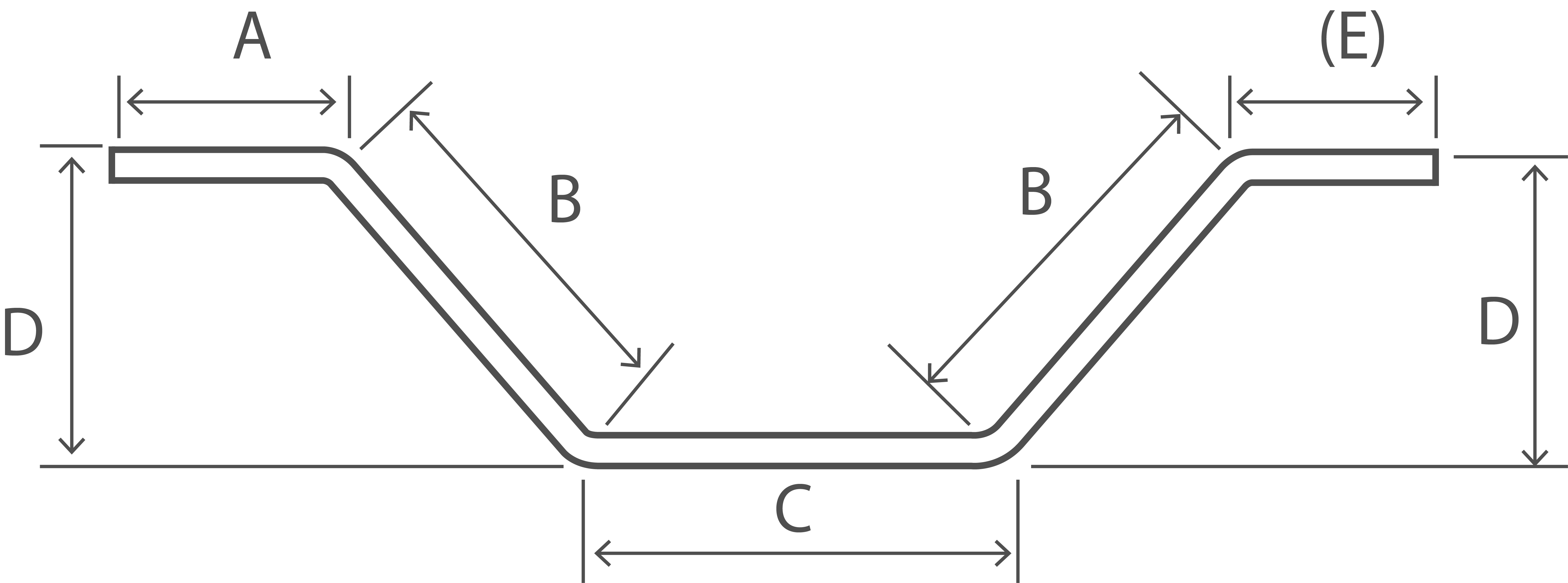A + 2B + C + (E) See Note 1

Shape Code 47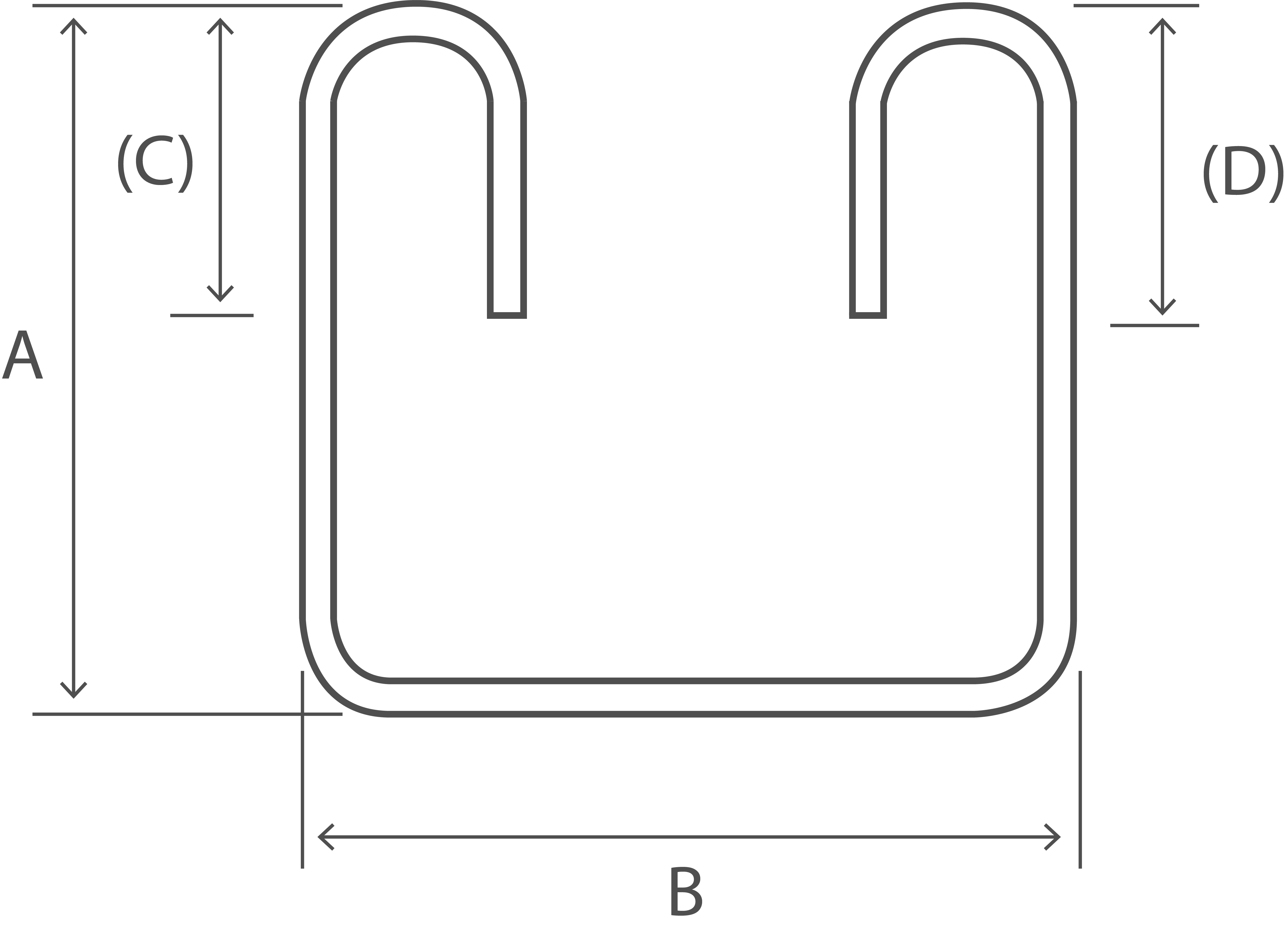2A + B + 2C + 1.5r - 3d (C) and (D) shall be equal and less than A Where (C) and (D) are to be minimized, the following formula may be used: L = 2A + 2B + max(16d, 160)

Shape Code 51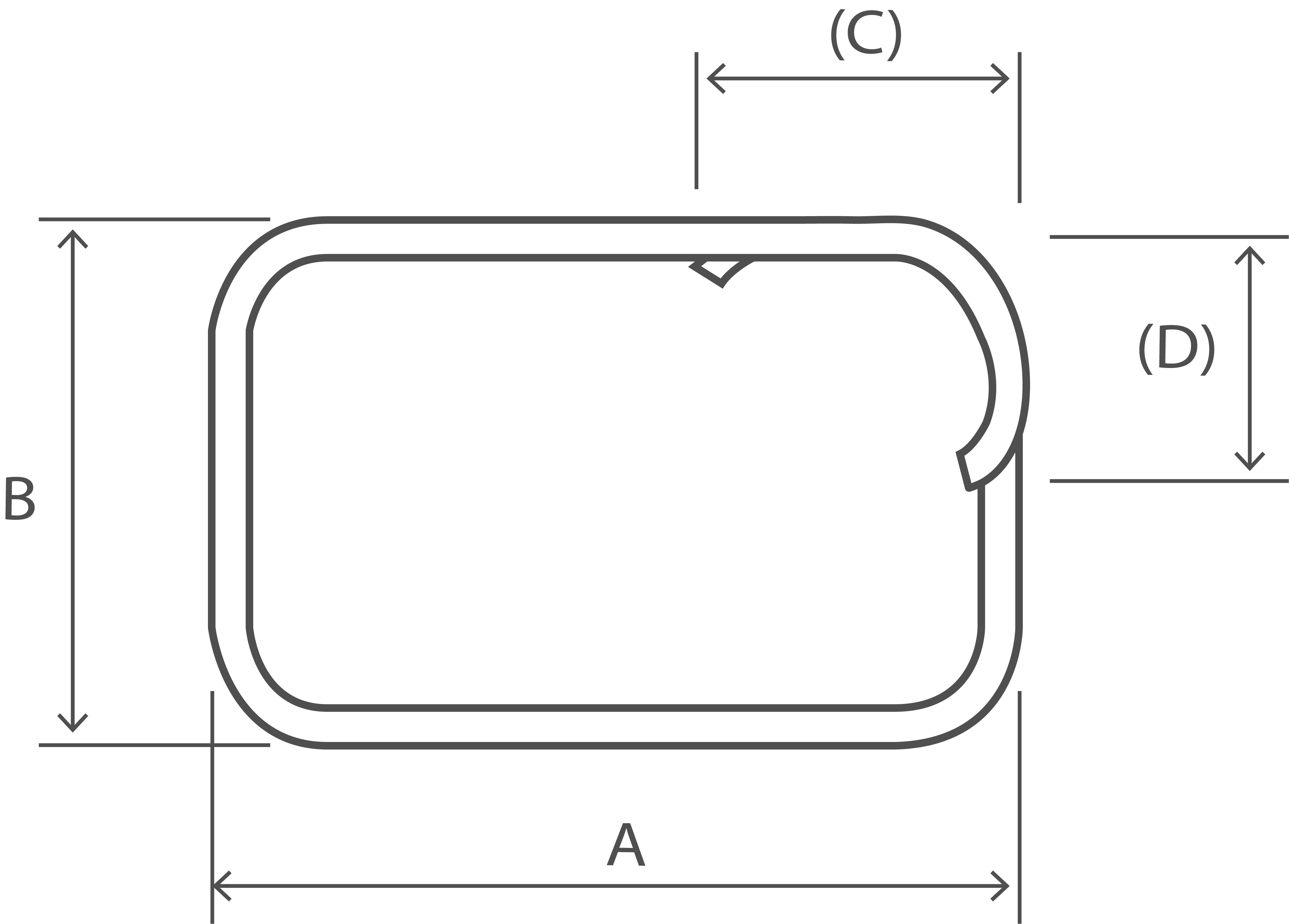2(A + B + (C)) - 2.5r - 5d (C) and (D) shall be equal and less than A and B Where (C) and (D) are to be minimized, the following formula may be used: L = 2A + 2B + max(16d, 160)

Shape Code 56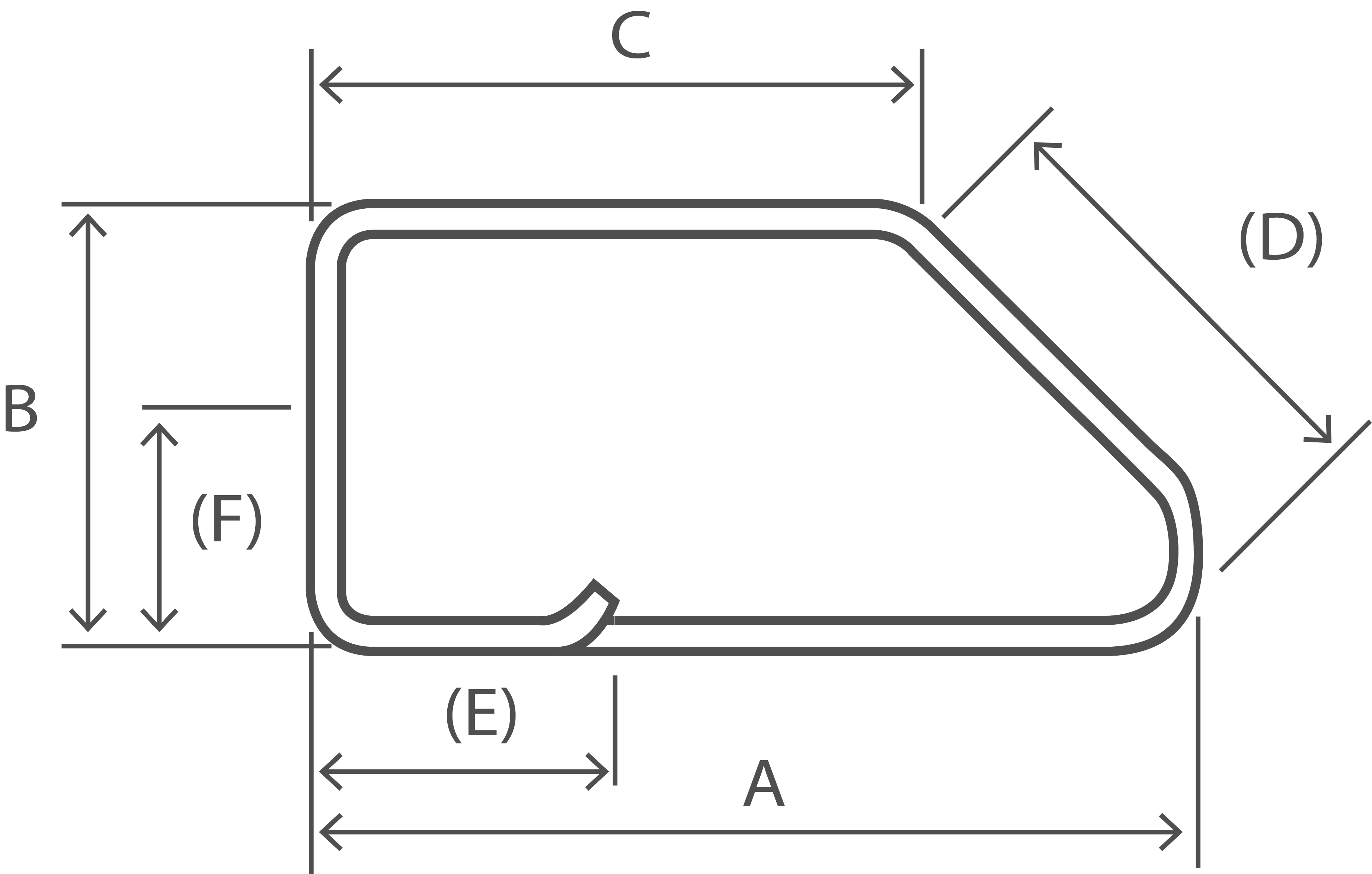A + B + C + (D) + 2(E) - 2.5r - 5d (E) and (F) shall be equal and not more than B and C

Shape Code 63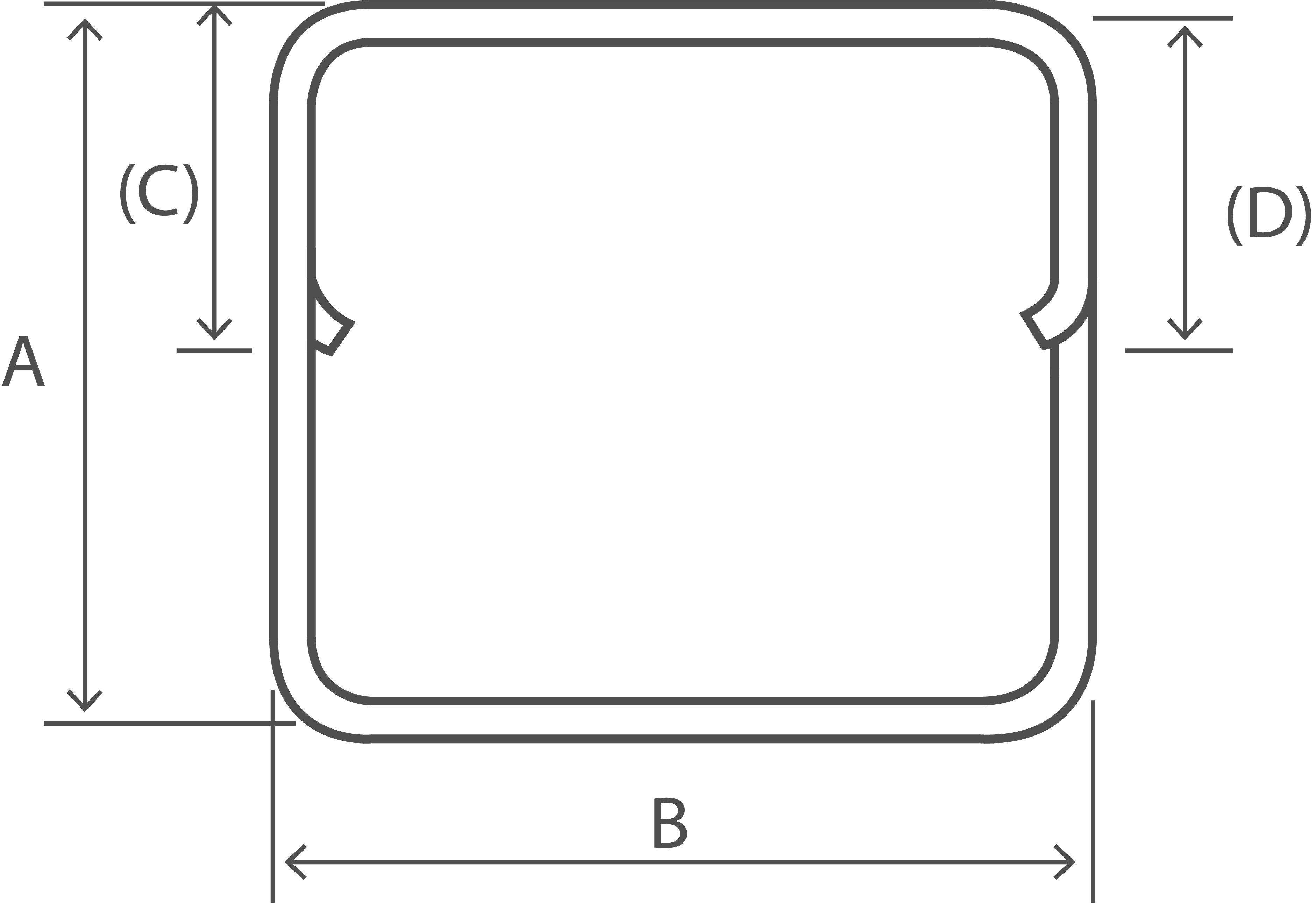2A + 3B + 2(C) -3r - 6d (C) and (D) shall be equal and less than A and B Where (C) and (D) are to be minimized, the following formula may be used: L = 2A + 3B + max(14d, 150)

Shape Code 64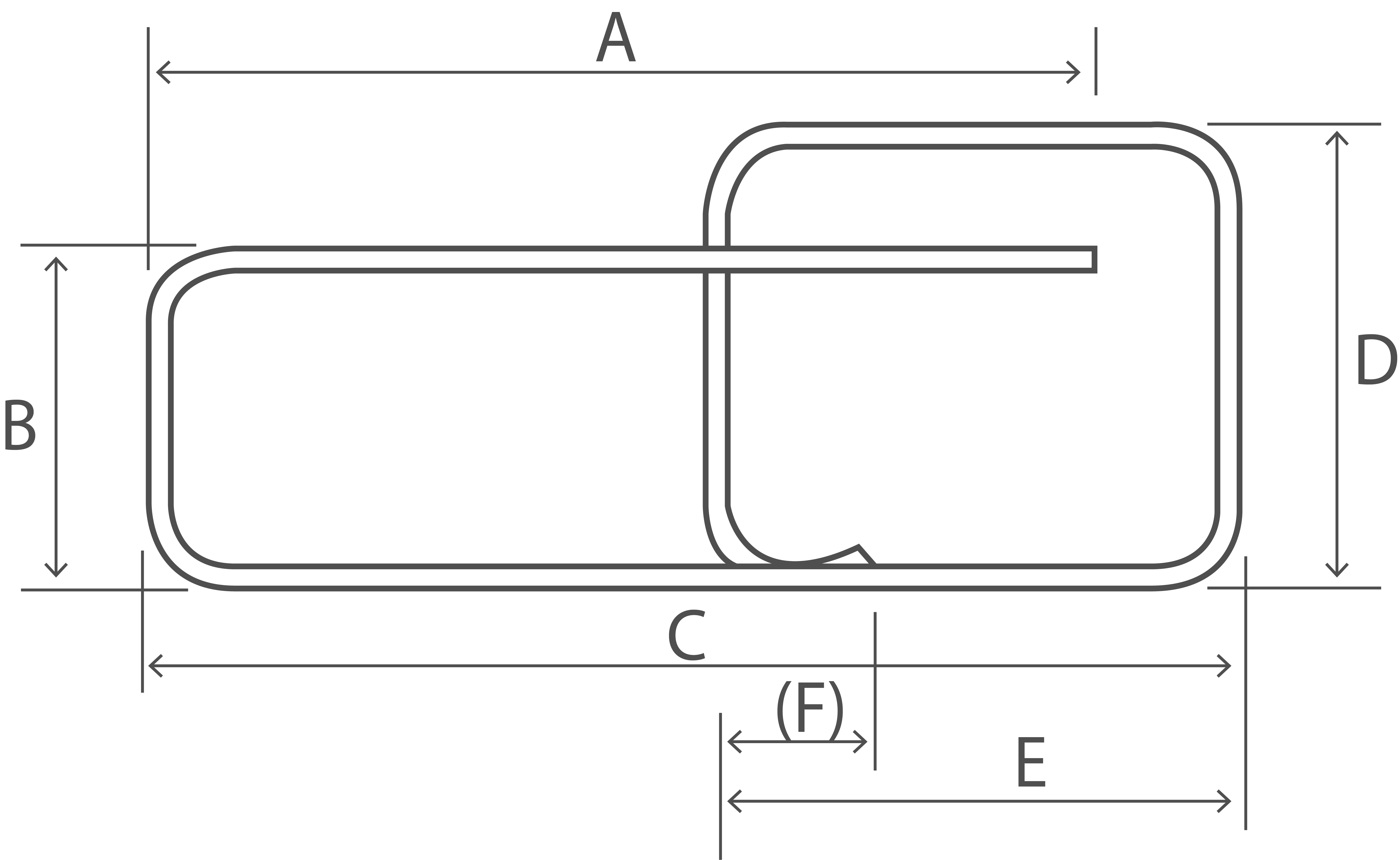A + B + C + 2D + E + (F) - 3r - 6d See Note 2

Shape Code 67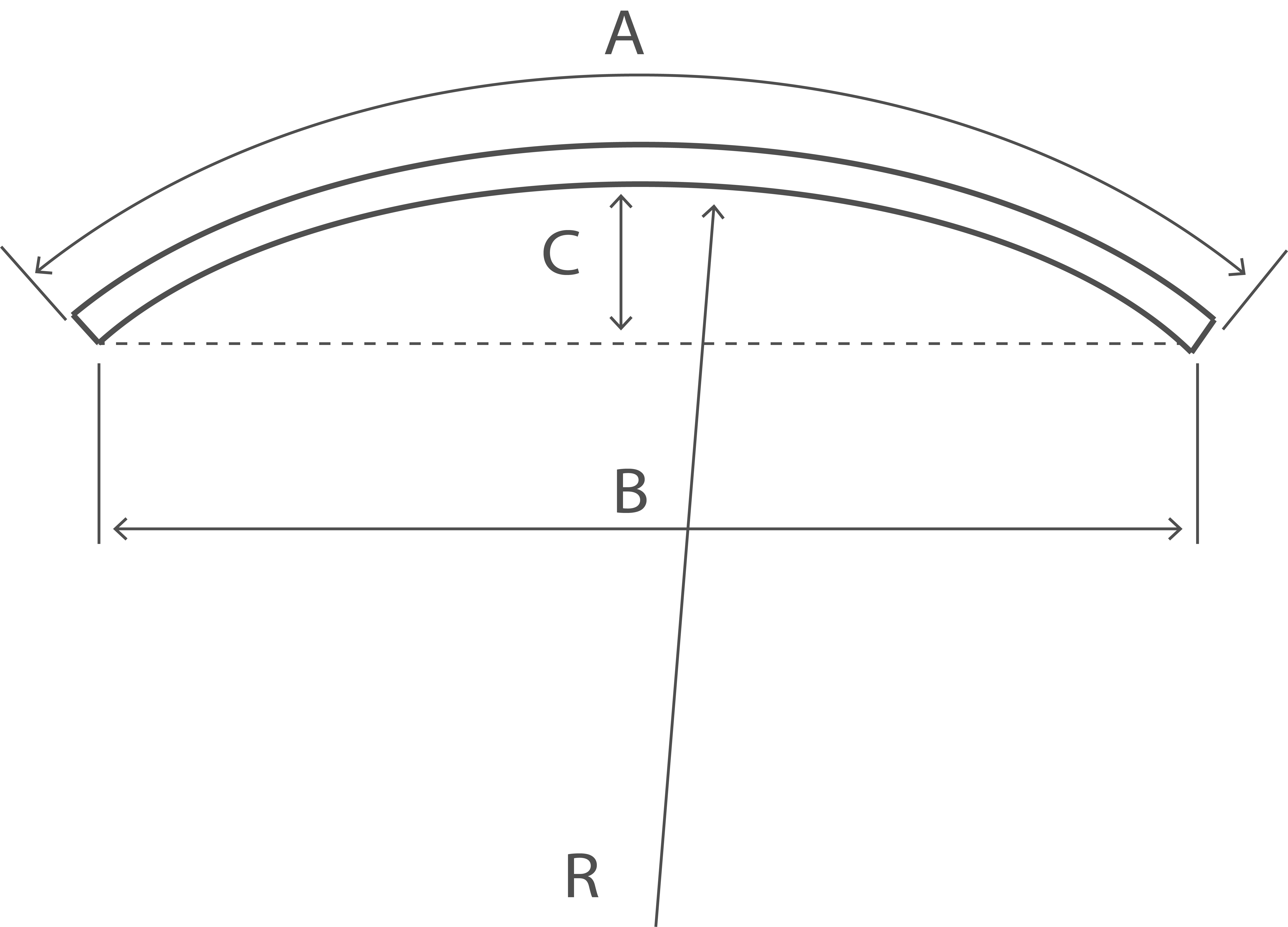A

Shape Code 75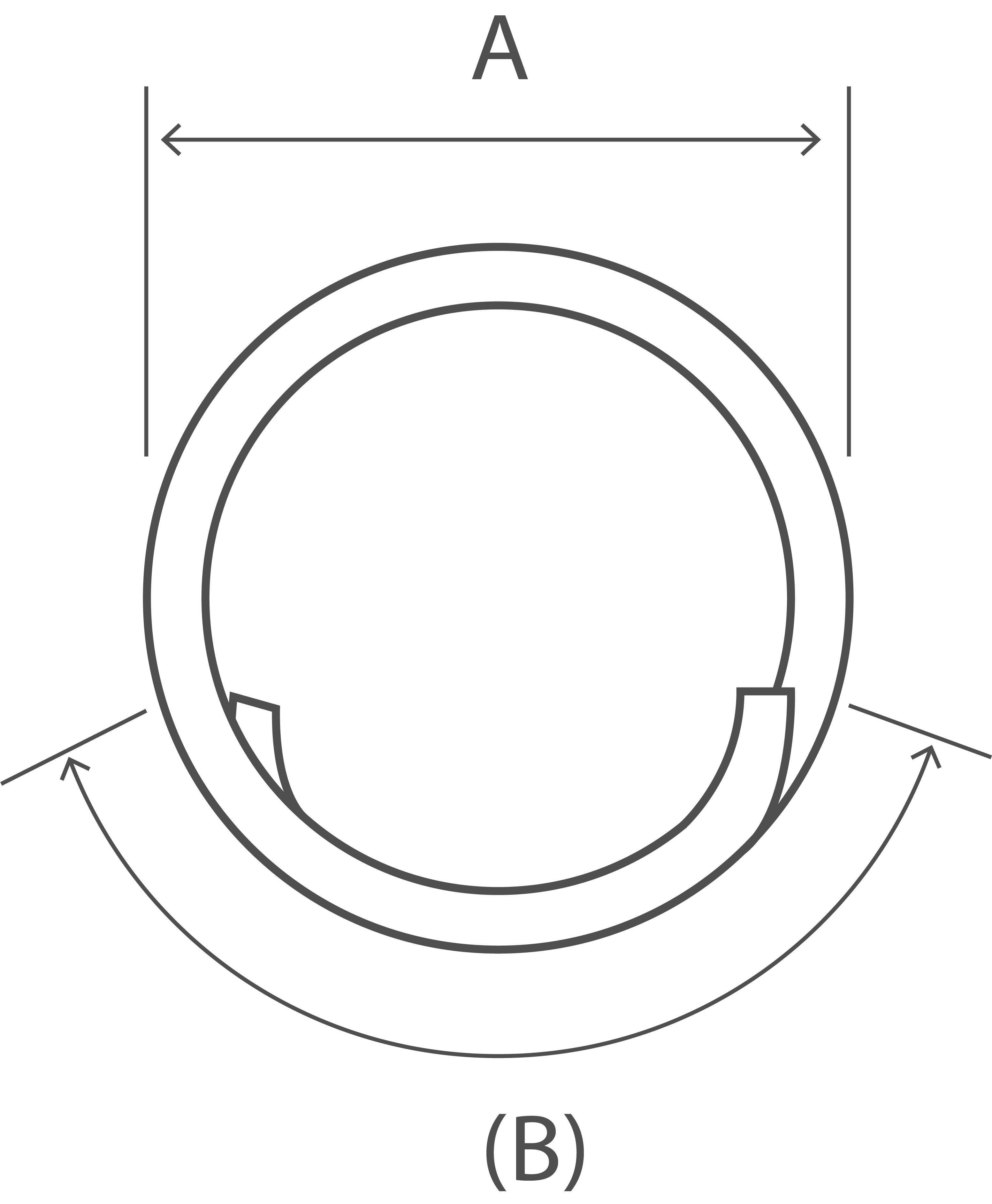π(A - d) + B Where B is the lap

Shape Code 77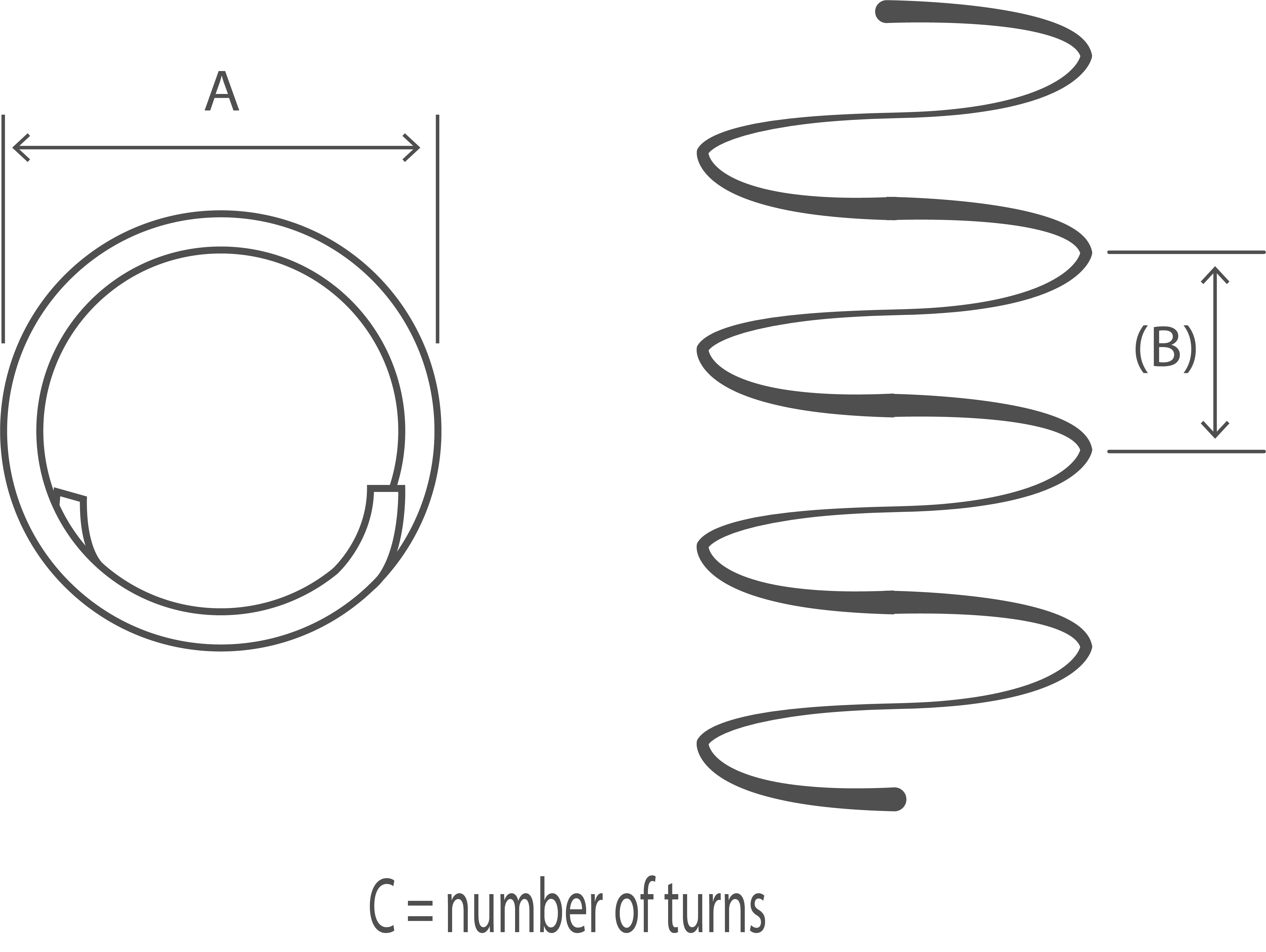Cπ(A - d) Where B is greater than A/5 this equation no longer applies in which case the following formula may be used: L = C((π(A - d))2 + B2)0.5

Shape Code 98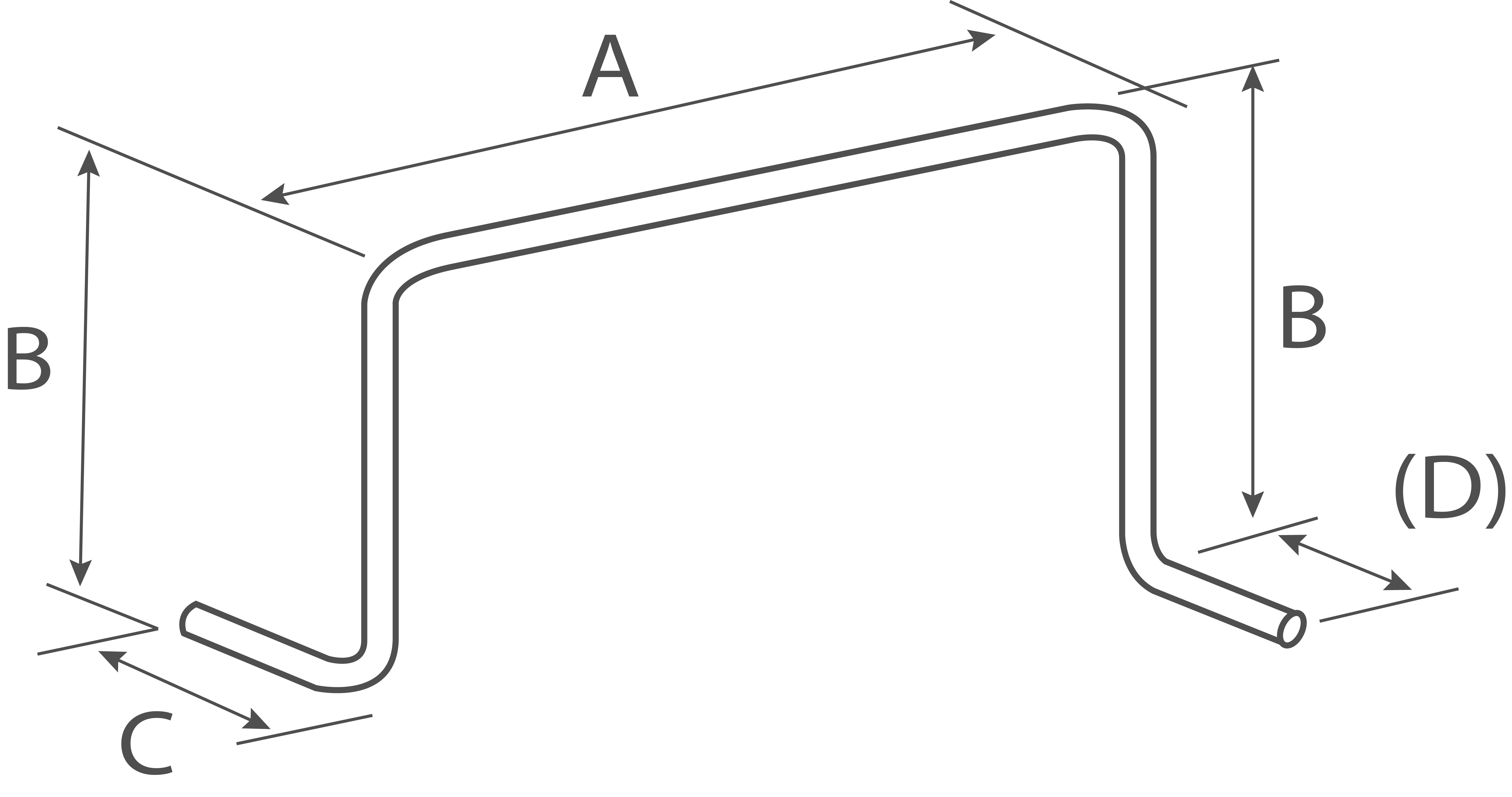A + 2B + C + (D) -2r - 4d Isometric sketch

Shape Code 99 All other shapes where standard shapes cannot be used. No other shape code number, form of designation or abbreviation shall be used in scheduling A dimensioned sketch shall be drawn over the dimension columns A to E. Every dimension shall be specified and the dimension that is to allow for permissible deviations shall be indicated in parenthesis, otherwise the fabricator is free to choose which dimension shall allow for tolerance To be calculated See Note 2.

 The values for minimum radius and end projection, r and P respectively (Minimum radius for scheduling and Minimum end projection) shall apply to all shape codes. The Dimensions in parentheses are the free dimensions, If a shape given in this table is required but a different dimension is to allow for the prossible deviations, the shape shall be drawn out and given the shape code 99 and the free dimension shall be indicated in parenthesis. The length of straight between two bends shall be at least 4d, where d is the diameter of the bar. NOTE 1 - The length equations for shape codes 14, 15, 25, 26, 27, 28, 29, 34, 35, 36, and 46 are approximate and where the bend angle is greater than 45°, the length should be calculated more accurately allowing for the difference between the specified overall dimensions and the true length measured along the central axis of the bar. When the bending angles approach 90°, it is preferable to specify shape code 99 with a fully dimensioned sketch. NOTE 2 - Five bends or more might be impractical within permitted tolerances. NOTE 3 - For shapes with straight and curved lengths (e.g. shape codes 12, 13, 22, 33, and 47) the largest practical mandrel size for the production of a continuous curve is 400 mm. NOTE 4 - Stock lengths are available in a limited number of lengths (e.g. 6m, 12m). Dimension A for shape code 01 should be regarded as indicative and used for the purpose of calculating total length. Actual delivery lengths should be by agreement with the supplier. Also, tolerances for shape code 01, stock lengths, shall be subject to the relevant product standard, e.g. BS 4449:2005.
Source

BSI British Standard documentation